# 4 Classification with Trees and Linear Models

These lecture notes are distributed in the hope that they will be useful. Any bug reports are appreciated.

## 4.1 Introduction

Let $$\mathbf{X}\in\mathbb{R}^{n\times p}$$ be an input matrix that consists of $$n$$ points in a $$p$$-dimensional space (each of the $$n$$ objects is described by means of $$p$$ numerical features)

Recall that in supervised learning, with each $$\mathbf{x}_{i,\cdot}$$ we associate the desired output $$y_i$$.

Hence, our dataset is $$[\mathbf{X}\ \mathbf{y}]$$ – where each object is represented as a row vector $$[\mathbf{x}_{i,\cdot}\ y_i]$$, $$i=1,\dots,n$$:

$[\mathbf{X}\ \mathbf{y}]= \left[ \begin{array}{ccccc} x_{1,1} & x_{1,2} & \cdots & x_{1,p} & y_1\\ x_{2,1} & x_{2,2} & \cdots & x_{2,p} & y_2\\ \vdots & \vdots & \ddots & \vdots & \vdots\\ x_{n,1} & x_{n,2} & \cdots & x_{n,p} & y_n\\ \end{array} \right].$

In this chapter we are still interested in classification tasks; we assume that each $$y_i$$ is a descriptive label.

Let’s assume that we are faced with binary classification tasks.

Hence, there are only two possible labels that we traditionally denote with $$0$$s and $$1$$s.

For example:

0 1
no yes
false true
failure success
healthy ill

Let’s recall the synthetic 2D dataset from the previous chapter (true decision boundary is at $$X_1=0$$), see Figure 4.1.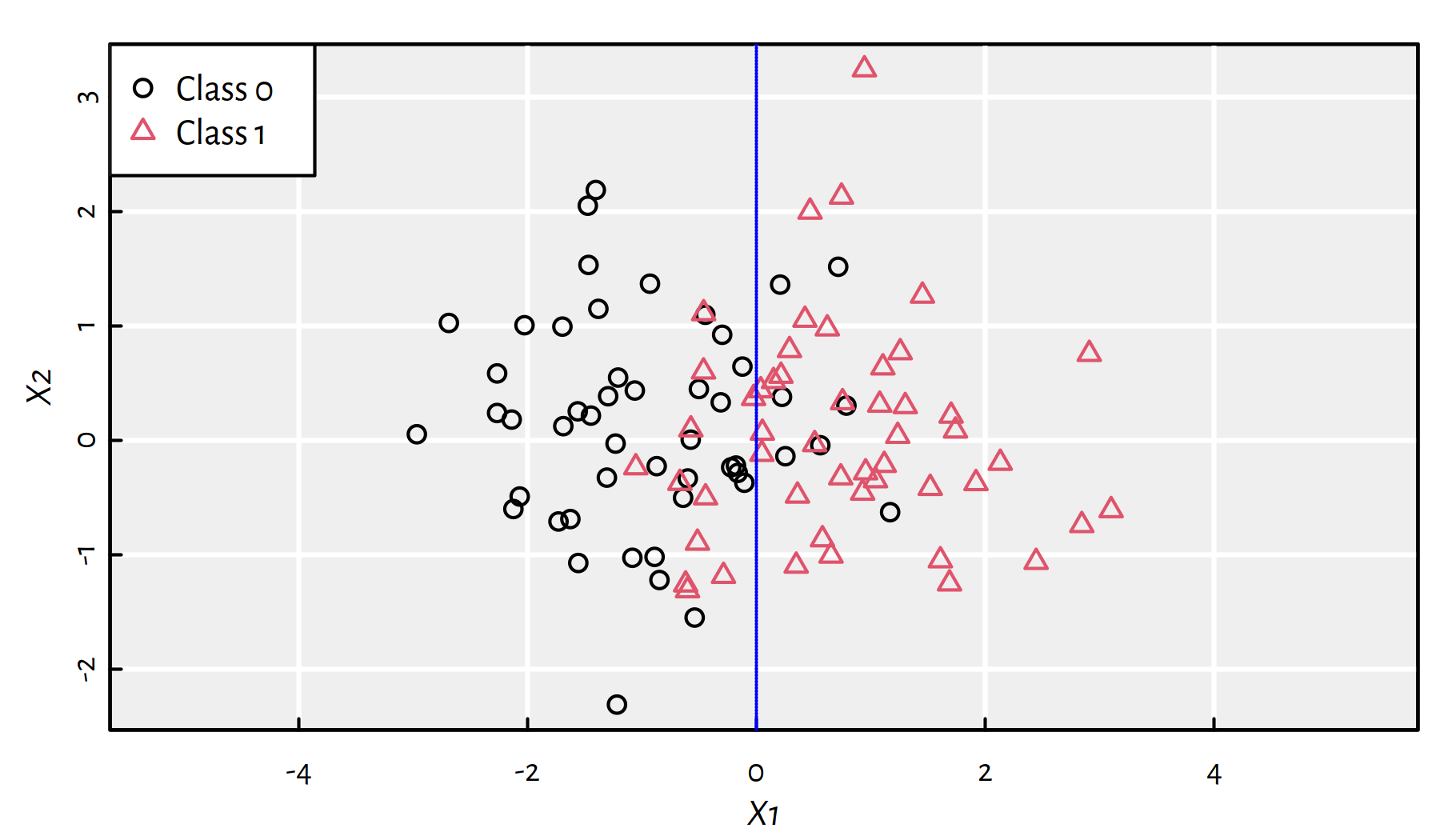Figure 4.1: A synthetic 2D dataset with the true decision boundary at $$X_1=0$$

### 4.1.2 Data

For illustration, we’ll be considering the Wine Quality dataset (white wines only):

wines <- read.csv("datasets/winequality-all.csv",
comment.char="#")
wines <- wines[wines$color == "white",] (n <- nrow(wines)) # number of samples ##  4898 The input matrix $$\mathbf{X}\in\mathbb{R}^{n\times p}$$ consists of the first 10 numeric variables: X <- as.matrix(wines[,1:10]) dim(X) ##  4898 10 head(X, 2) # first two rows ## fixed.acidity volatile.acidity citric.acid residual.sugar ## 1600 7.0 0.27 0.36 20.7 ## 1601 6.3 0.30 0.34 1.6 ## chlorides free.sulfur.dioxide total.sulfur.dioxide density pH ## 1600 0.045 45 170 1.001 3.0 ## 1601 0.049 14 132 0.994 3.3 ## sulphates ## 1600 0.45 ## 1601 0.49 The 11th variable measures the amount of alcohol (in %). We will convert this dependent variable to a binary one: • 0 == (alcohol < 12) == lower-alcohol wines • 1 == (alcohol >= 12) == higher-alcohol wines # recall that TRUE == 1 Y <- factor(as.character(as.numeric(wines$alcohol >= 12)))
table(Y)
## Y
##    0    1
## 4085  813

60/40% train-test split:

set.seed(123) # reproducibility matters
random_indices <- sample(n)
head(random_indices) # preview
##  2463 2511 2227  526 4291 2986
# first 60% of the indices (they are arranged randomly)
# will constitute the train sample:
train_indices <- random_indices[1:floor(n*0.6)]
X_train <- X[train_indices,]
Y_train <- Y[train_indices]
# the remaining indices (40%) go to the test sample:
X_test  <- X[-train_indices,]
Y_test  <- Y[-train_indices]

Let’s also compute Z_train and Z_test, being the standardised versions of X_train and X_test, respectively.

means <- apply(X_train, 2, mean) # column means
sds   <- apply(X_train, 2, sd)   # column standard deviations
Z_train <- t(apply(X_train, 1, function(r) (r-means)/sds))
Z_test  <- t(apply(X_test,  1, function(r) (r-means)/sds))
get_metrics <- function(Y_pred, Y_test)
{
C <- table(Y_pred, Y_test) # confusion matrix
stopifnot(dim(C) == c(2, 2))
c(Acc=(C[1,1]+C[2,2])/sum(C), # accuracy
Prec=C[2,2]/(C[2,2]+C[2,1]), # precision
Rec=C[2,2]/(C[2,2]+C[1,2]), # recall
F=C[2,2]/(C[2,2]+0.5*C[1,2]+0.5*C[2,1]), # F-measure
# Confusion matrix items:
TN=C[1,1], FN=C[1,2],
FP=C[2,1], TP=C[2,2]
) # return a named vector
}

Let’s go back to the K-NN algorithm.

library("FNN")
Y_knn5   <- knn(X_train, X_test, Y_train, k=5)
Y_knn9   <- knn(X_train, X_test, Y_train, k=9)
Y_knn5s  <- knn(Z_train, Z_test, Y_train, k=5)
Y_knn9s  <- knn(Z_train, Z_test, Y_train, k=9)

Recall the quality metrics we have obtained previously (as a point of reference):

cbind(
Knn5=get_metrics(Y_knn5, Y_test),
Knn9=get_metrics(Y_knn9, Y_test),
Knn5s=get_metrics(Y_knn5s, Y_test),
Knn9s=get_metrics(Y_knn9s, Y_test)
)
##            Knn5       Knn9      Knn5s      Knn9s
## Acc     0.81735    0.81939    0.91429    0.91378
## Prec    0.38674    0.34959    0.82251    0.83636
## Rec     0.22082    0.13565    0.59937    0.58044
## F       0.28112    0.19545    0.69343    0.68529
## TN   1532.00000 1563.00000 1602.00000 1607.00000
## FN    247.00000  274.00000  127.00000  133.00000
## FP    111.00000   80.00000   41.00000   36.00000
## TP     70.00000   43.00000  190.00000  184.00000

In this chapter we discuss the following simple and educational (yet practically useful) classification algorithms:

• decision trees,
• binary logistic regression.

## 4.2 Decision Trees

### 4.2.1 Introduction

Note that a K-NN classifier discussed in the previous chapter is model-free. The whole training set must be stored and referred to at all times.

Therefore, it doesn’t explain the data we have – we may use it solely for the purpose of prediction.

Perhaps one of the most interpretable (and hence human-friendly) models consist of decision rules of the form:

IF $$x_{i,j_1}\le v_1$$ AND … AND $$x_{i,j_r}\le v_r$$ THEN $$\hat{y}_i=1$$.

These can be organised into a hierarchy for greater readability.

This idea inspired the notion of decision trees .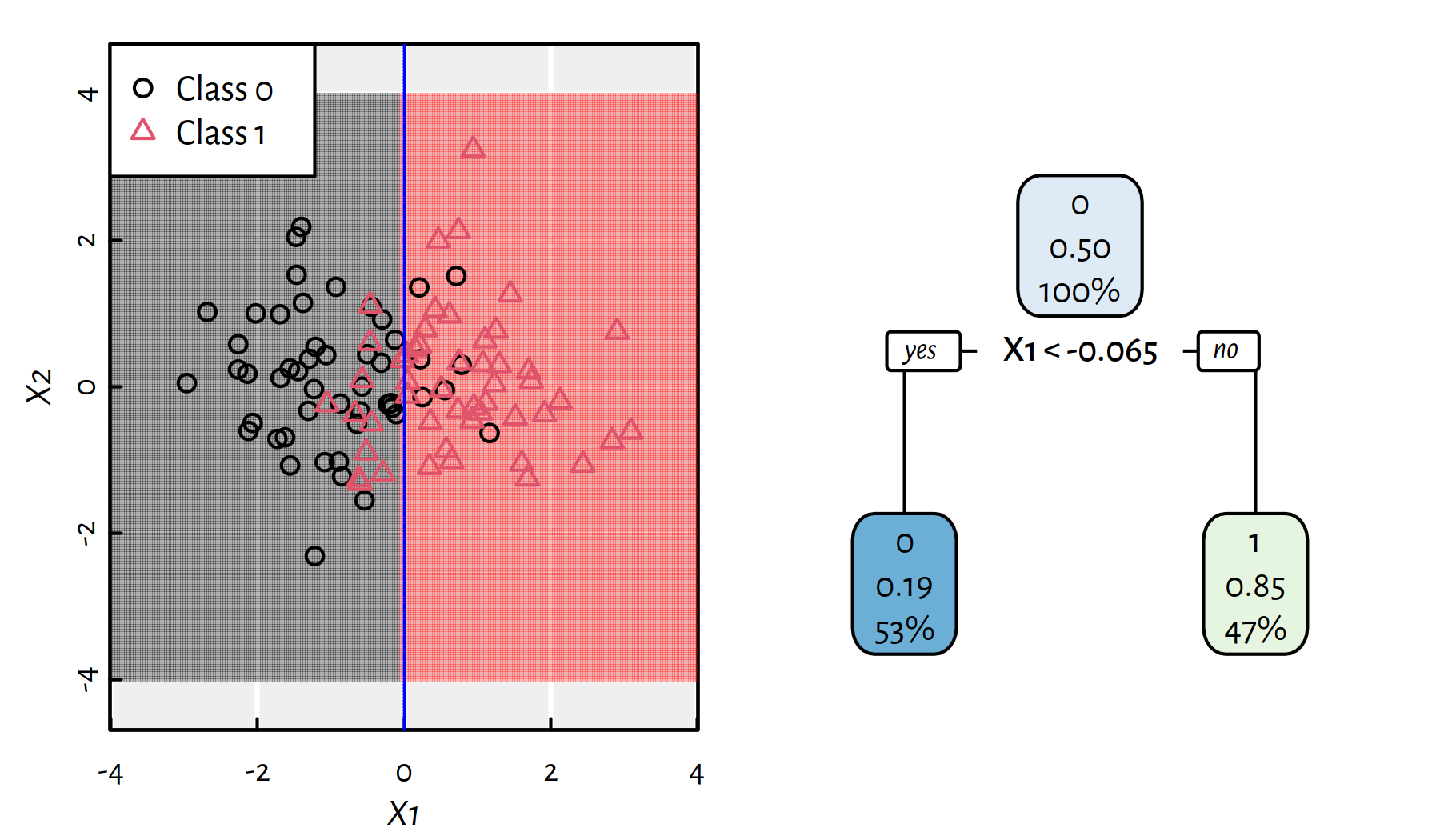Figure 4.2: The simplest decision tree for the synthetic 2D dataset and the corresponding decision boundaries

Figure 4.2 depicts a very simple decision tree for the aforementioned synthetic dataset. There is only one decision boundary (based on $$X_1$$) that splits data into the “left” and “right” sides. Each tree node reports 3 pieces of information:

• dominating class (0 or 1)
• (relative) proportion of 1s represented in a node
• (absolute) proportion of all observations in a node

Figures 4.3 and 4.4 depict trees with more decision rules. Take a moment to contemplate how the corresponding decision boundaries changed with the introduction of new decision rules.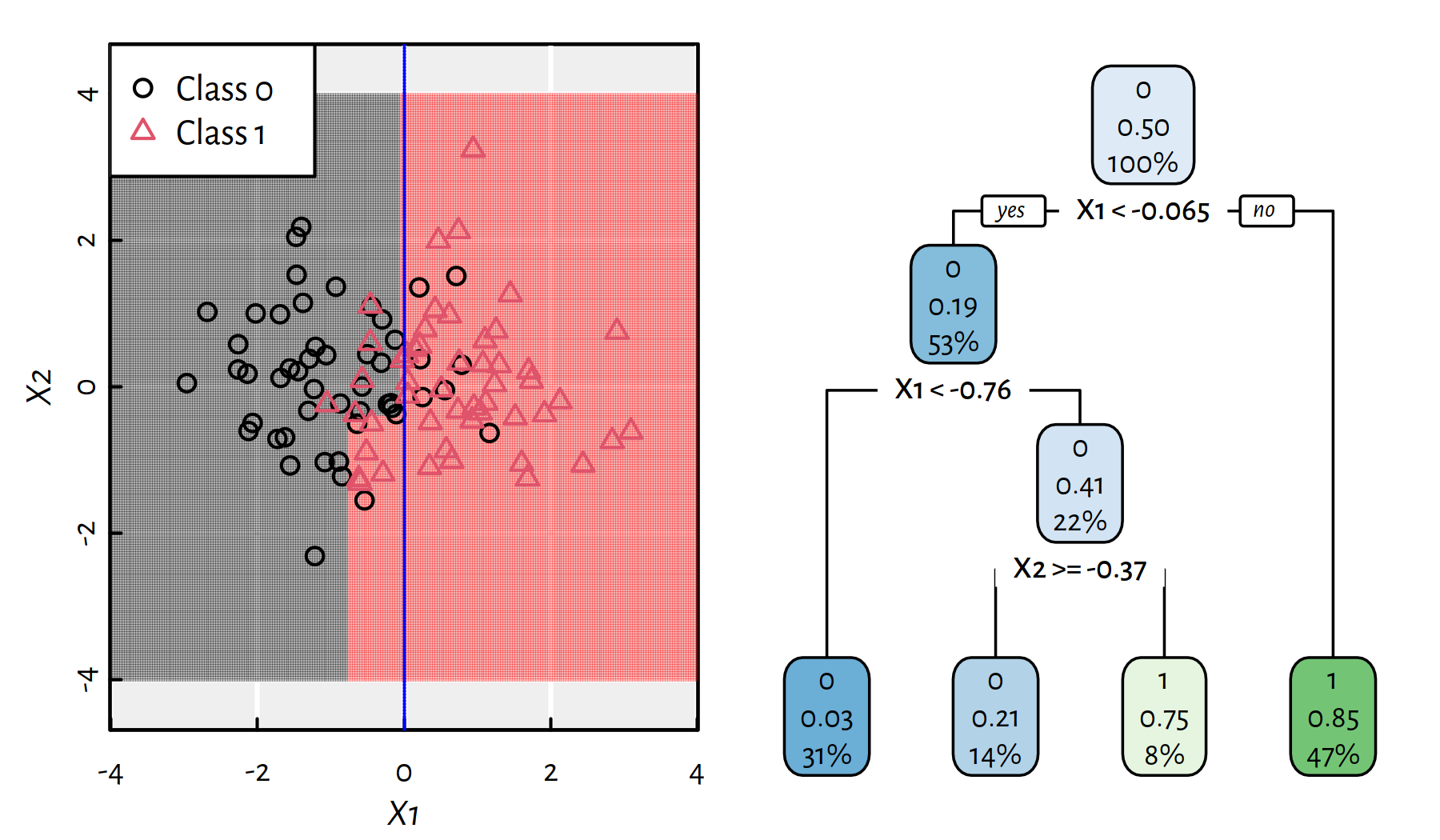Figure 4.3: A more complicated decision tree for the synthetic 2D dataset and the corresponding decision boundaries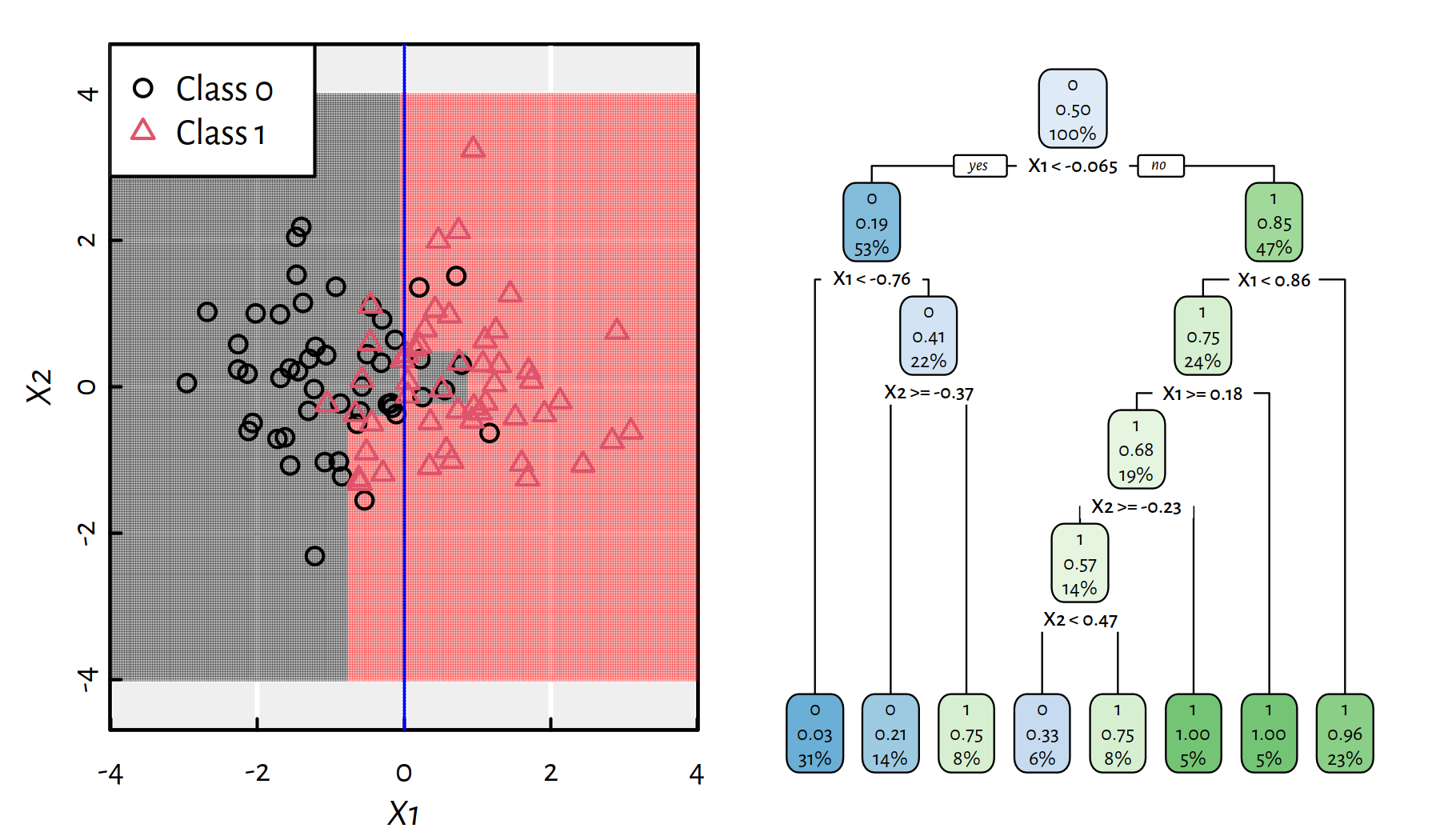Figure 4.4: An even more complicated decision tree for the synthetic 2D dataset and the corresponding decision boundaries

### 4.2.2 Example in R

We will use the rpart() function from the rpart package to build a classification tree.

library("rpart")
library("rpart.plot")
set.seed(123)

rpart() uses a formula (~) interface, hence it will be easier to feed it with data in a data.frame form.

XY_train <- cbind(as.data.frame(X_train), Y=Y_train)
XY_test <- cbind(as.data.frame(X_test), Y=Y_test)

Fit and plot a decision tree, see Figure 4.5.

t1 <- rpart(Y~., data=XY_train, method="class")
rpart.plot(t1, tweak=1.1, fallen.leaves=FALSE, digits=3)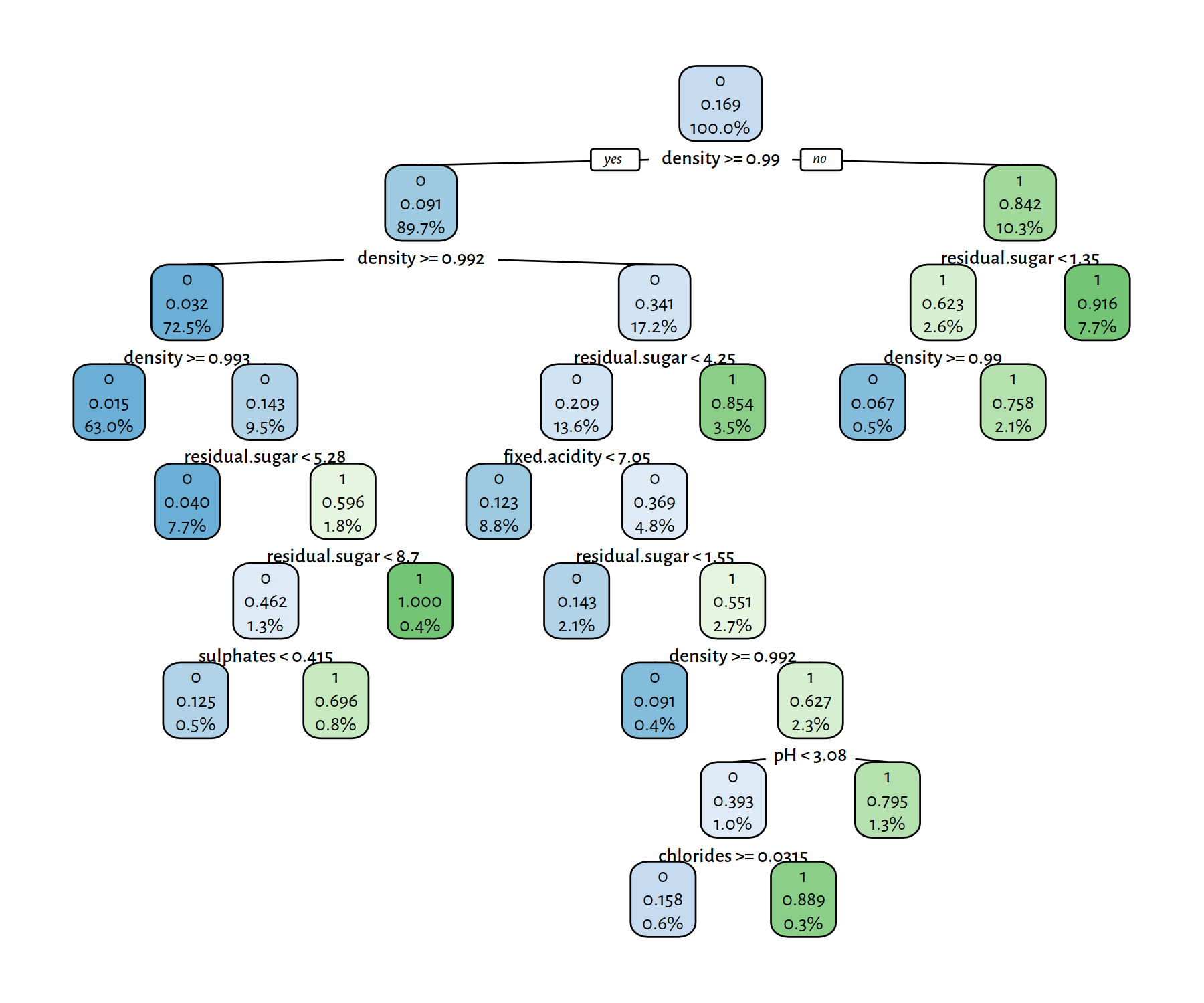Figure 4.5: A decision tree for the wines dataset

We can build less or more complex trees by playing with the cp parameter, see Figures 4.6 and 4.7.

# cp = complexity parameter, smaller → more complex tree
t2 <- rpart(Y~., data=XY_train, method="class", cp=0.1)
rpart.plot(t2, tweak=1.1, fallen.leaves=FALSE, digits=3)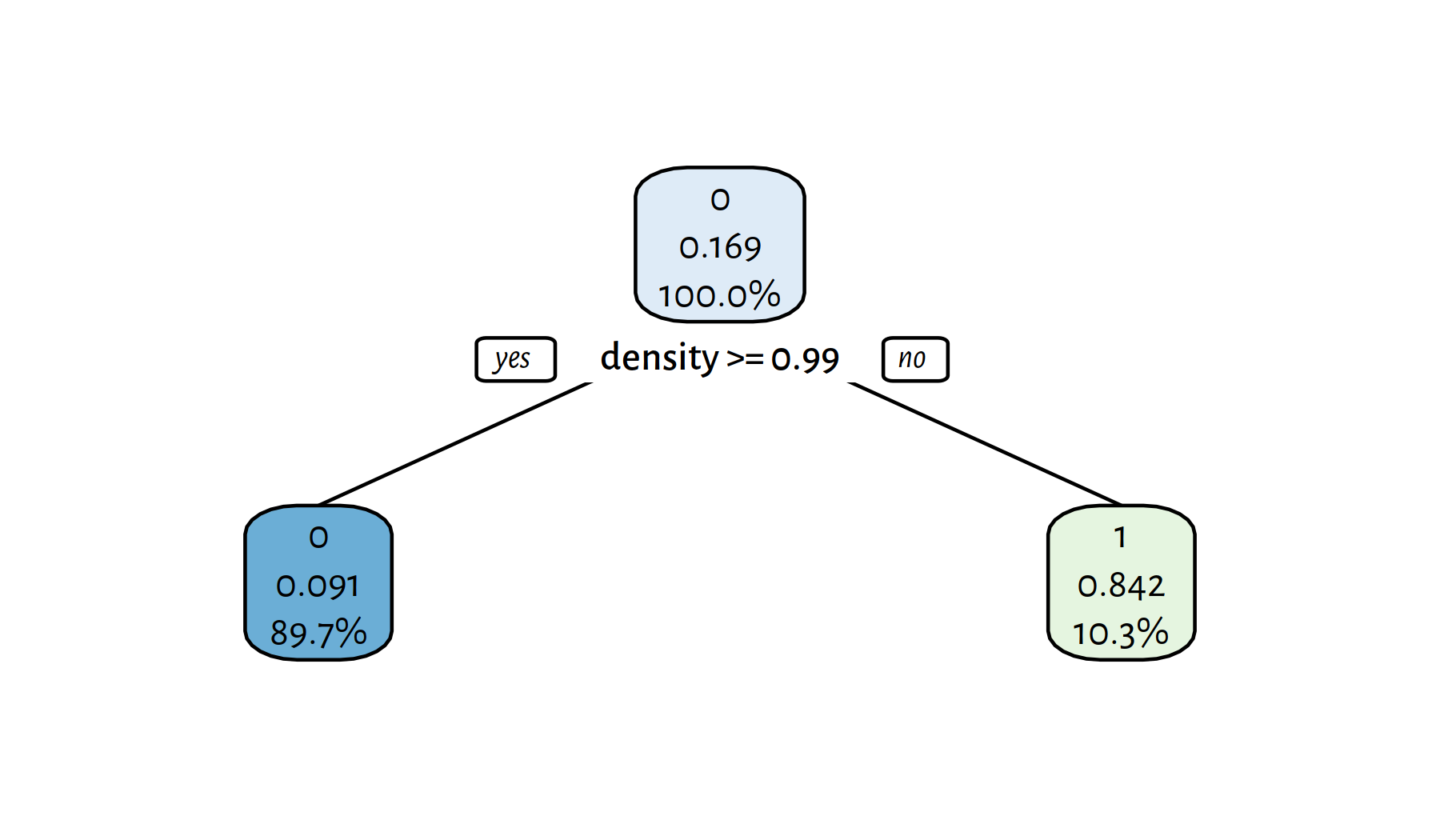Figure 4.6: A (simpler) decision tree for the wines dataset

# cp = complexity parameter, smaller → more complex tree
t3 <- rpart(Y~., data=XY_train, method="class", cp=0.00001)
rpart.plot(t3, tweak=1.1, fallen.leaves=FALSE, digits=3)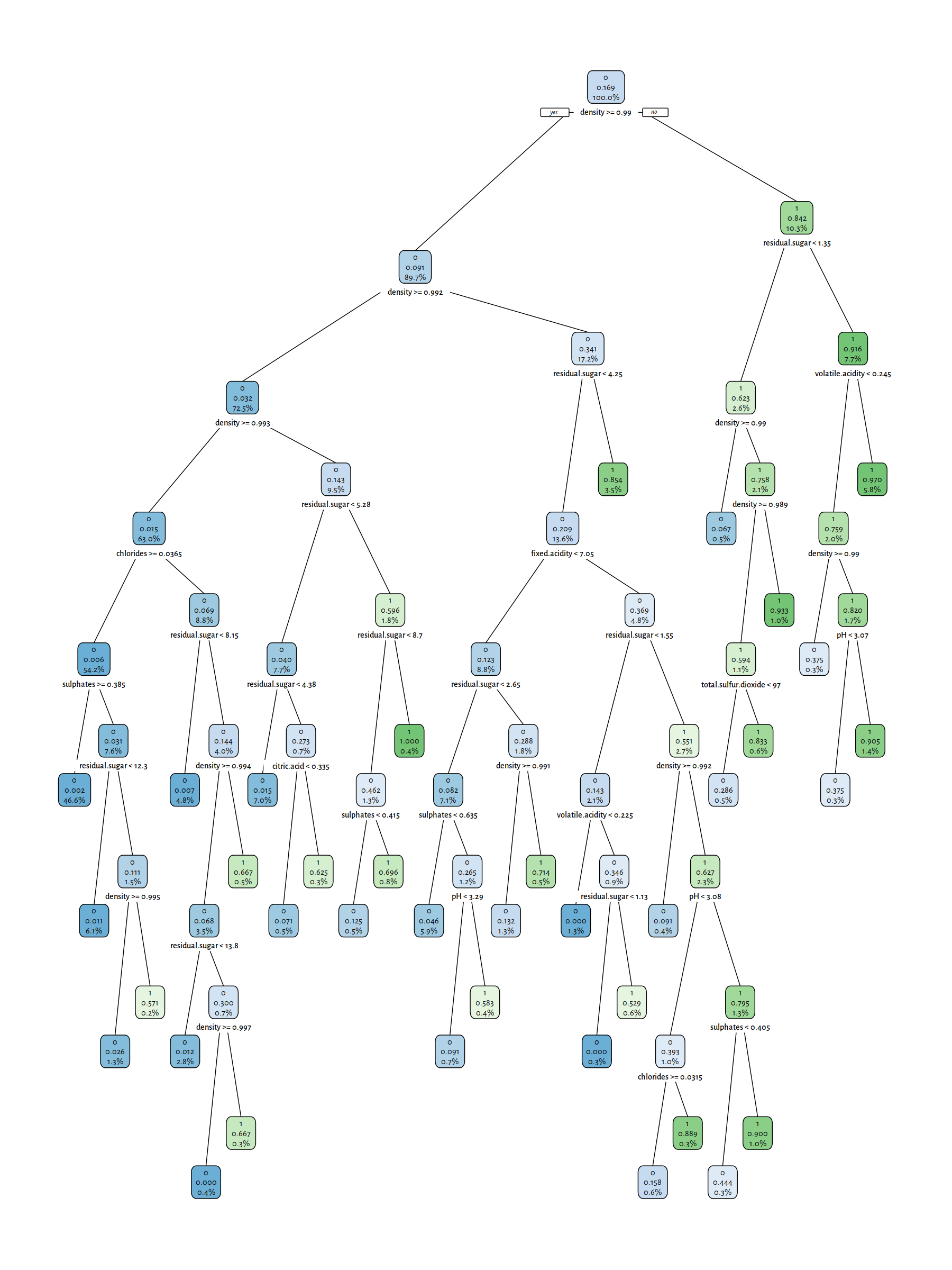Figure 4.7: A (more complex) decision tree for the wines dataset

Trees with few decision rules actually are very nicely interpretable. On the other hand, plotting of the complex ones is just hopeless; we should treat them as “black boxes” instead.

Let’s make some predictions:

Y_pred <- predict(t1, XY_test, type="class")
get_metrics(Y_pred, Y_test)
##        Acc       Prec        Rec          F         TN         FN
##    0.92857    0.80623    0.73502    0.76898 1587.00000   84.00000
##         FP         TP
##   56.00000  233.00000
Y_pred <- predict(t2, XY_test, type="class")
get_metrics(Y_pred, Y_test)
##        Acc       Prec        Rec          F         TN         FN
##    0.90255    0.83871    0.49211    0.62028 1613.00000  161.00000
##         FP         TP
##   30.00000  156.00000
Y_pred <- predict(t3, XY_test, type="class")
get_metrics(Y_pred, Y_test)
##        Acc       Prec        Rec          F         TN         FN
##    0.91837    0.73433    0.77603    0.75460 1554.00000   71.00000
##         FP         TP
##   89.00000  246.00000
Remark.

(*) Interestingly, rpart() also provides us with information about the importance degrees of each independent variable.

t1$variable.importance/sum(t1$variable.importance)
##              density       residual.sugar        fixed.acidity
##            0.6562490            0.1984221            0.0305167
##            chlorides                   pH     volatile.acidity
##            0.0215008            0.0209678            0.0192880
##            sulphates total.sulfur.dioxide          citric.acid
##            0.0184293            0.0140482            0.0119201
##  free.sulfur.dioxide
##            0.0086579

### 4.2.3 A Note on Decision Tree Learning

Learning an optimal decision tree is a computationally hard problem – we need some heuristics.

Examples:

• ID3 (Iterative Dichotomiser 3)
• C4.5 algorithm
• CART by Leo Breiman et al.,

(**) Decision trees are most often constructed by a greedy, top-down recursive partitioning, see., e.g., .

## 4.3 Binary Logistic Regression

### 4.3.1 Motivation

Recall that for a regression task, we fitted a very simple family of models – the linear ones – by minimising the sum of squared residuals.

This approach was pretty effective.

(Very) theoretically, we could treat the class labels as numeric $$0$$s and $$1$$s and apply regression models in a binary classification task.

XY_train_r <- cbind(as.data.frame(X_train),
Y=as.numeric(Y_train)-1 # 0.0 or 1.0
)
XY_test_r <- cbind(as.data.frame(X_test),
Y=as.numeric(Y_test)-1 # 0.0 or 1.0
)
f_r <- lm(Y~density+residual.sugar+pH, data=XY_train_r)
Y_pred_r <- predict(f_r, XY_test_r)
summary(Y_pred_r)
##    Min. 1st Qu.  Median    Mean 3rd Qu.    Max.
## -3.0468 -0.0211  0.1192  0.1645  0.3491  0.8892

The predicted outputs, $$\hat{Y}$$, are arbitrary real numbers, but we can convert them to binary ones by checking if, e.g., $$\hat{Y}>0.5$$.

Y_pred <- as.numeric(Y_pred_r>0.5)
round(get_metrics(Y_pred, XY_test_r$Y), 3) ## Acc Prec Rec F TN FN FP TP ## 0.927 0.865 0.647 0.740 1611.000 112.000 32.000 205.000 Remark. (*) The threshold $$T=0.5$$ could even be treated as a free parameter we optimise for (w.r.t. different metrics over the validation sample), see Figure 4.8.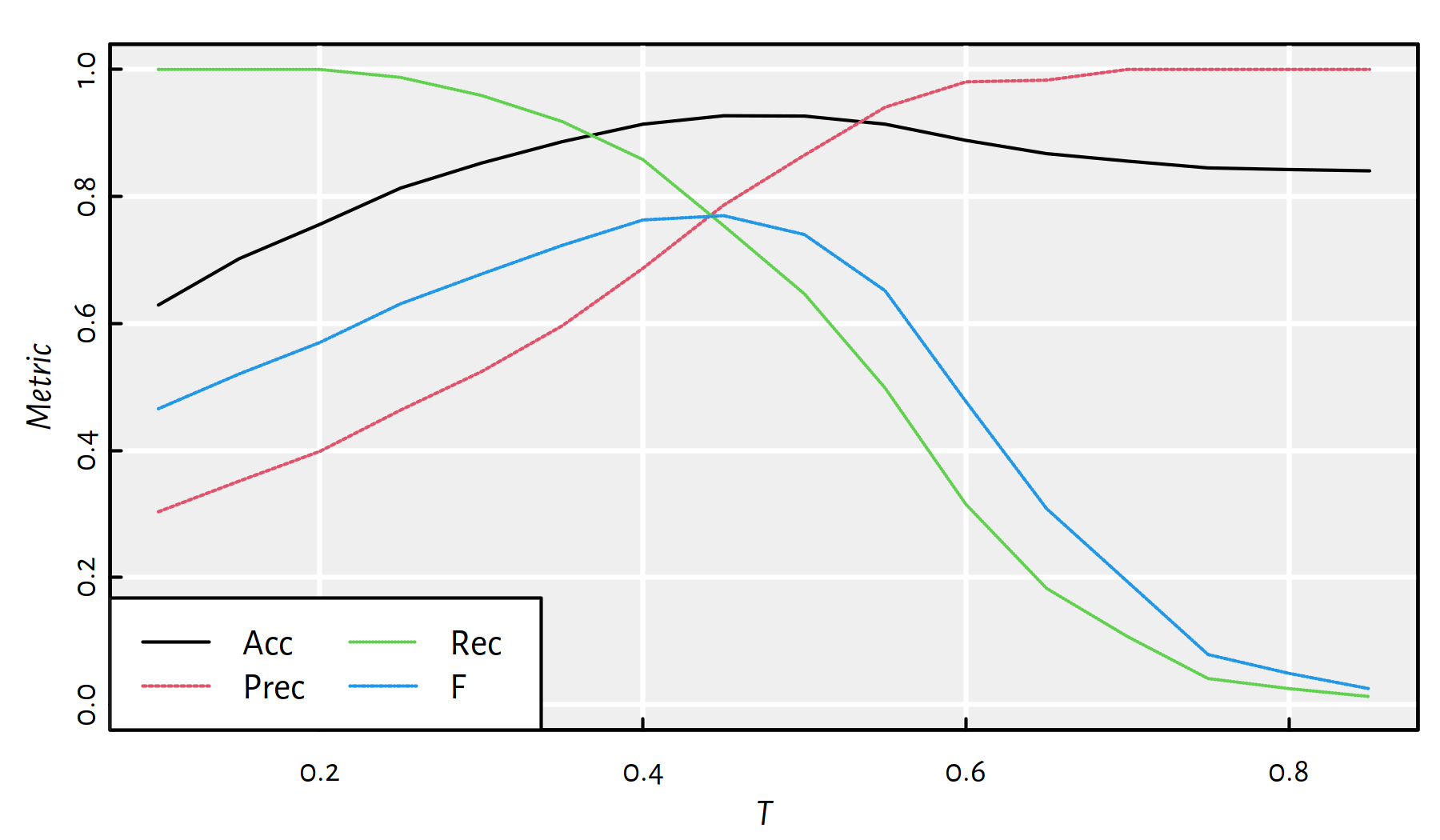Figure 4.8: Quality metrics for a binary classifier “Classify X as 1 if $$f(X)>T$$ and as 0 if $$f(X)\le T$$ Despite we can, we shouldn’t use linear regression for classification. Treating class labels “0” and “1” as ordinary real numbers just doesn’t cut it – we intuitively feel that we are doing something ugly. Luckily, there is a better, more meaningful approach that still relies on a linear model, but has the right semantics. ### 4.3.2 Logistic Model Inspired by this idea, we could try modelling the probability that a given point belongs to class $$1$$. This could also provide us with the confidence in our prediction. Probability is a number in $$[0,1]$$, but the outputs of a linear model are arbitrary real numbers. However, we could transform those real-valued outputs by means of some function $$\phi:\mathbb{R}\to[0,1]$$ (preferably S-shaped == sigmoid), so as to get: $\Pr(Y=1|\mathbf{X},\boldsymbol\beta)=\phi(\beta_0 + \beta_1 X_1 + \beta_2 X_2 + \dots + \beta_p X_p).$ Remark. The above reads as “Probability that $$Y$$ is from class 1 given $$\mathbf{X}$$ and $$\boldsymbol\beta$$”. A popular choice is the logistic sigmoid function, see Figure 4.9: $\phi(t) = \frac{1}{1+e^{-t}} = \frac{e^t}{1+e^t}.$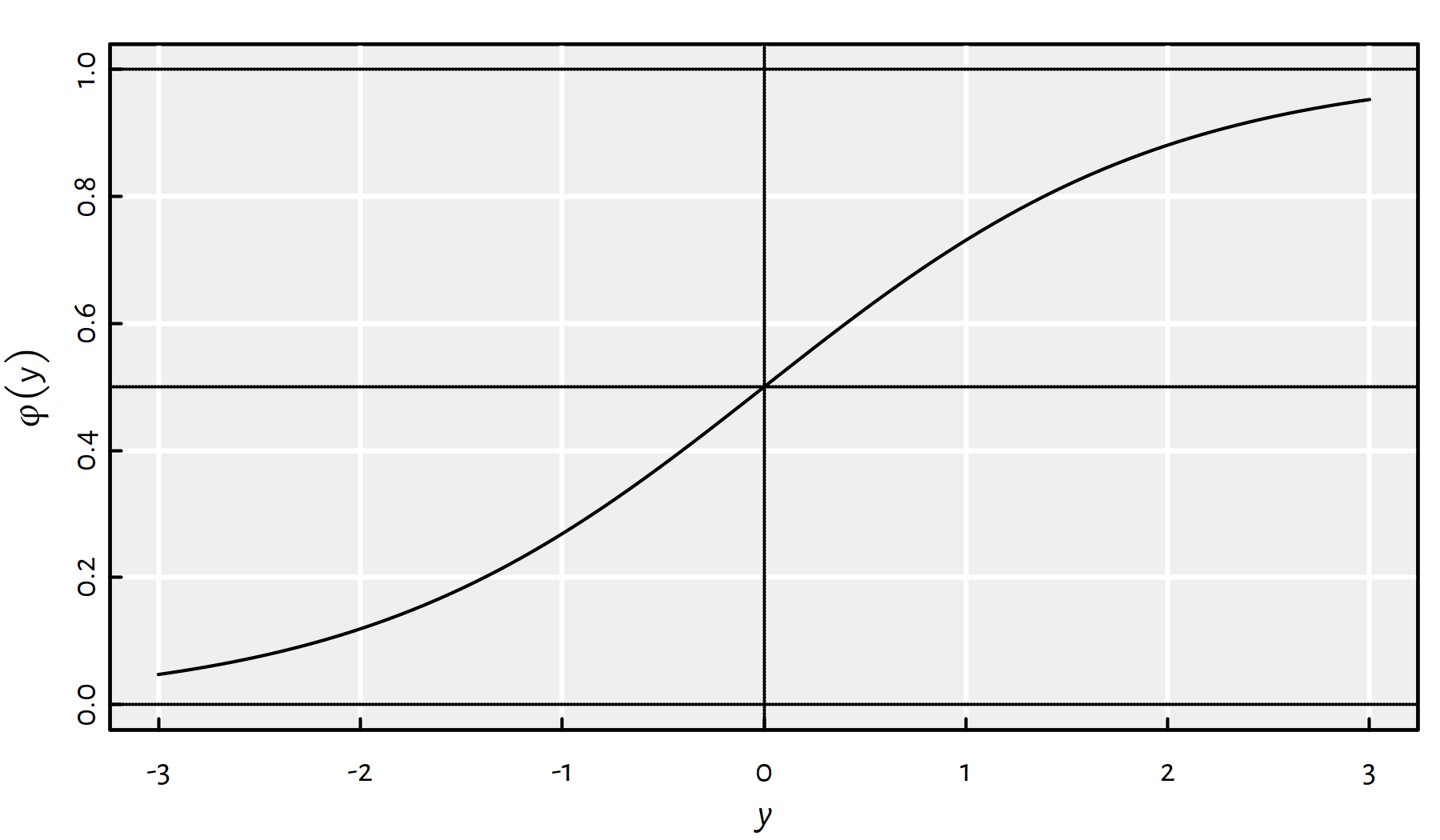Figure 4.9: The logistic sigmoid function, $$\varphi$$ Hence our model becomes: $Y=\frac{1}{1+e^{-(\beta_0 + \beta_1 X_1 + \beta_2 X_2 + \dots + \beta_p X_p)}}$ It is an instance of a generalised linear model (glm) (there are of course many other possible generalisations). ### 4.3.3 Example in R Let us first fit a simple (i.e., $$p=1$$) logistic regression model using the density variable. The goodness-of-fit measure used in this problem will be discussed a bit later. (f <- glm(Y~density, data=XY_train, family=binomial("logit"))) ## ## Call: glm(formula = Y ~ density, family = binomial("logit"), data = XY_train) ## ## Coefficients: ## (Intercept) density ## 1173 -1184 ## ## Degrees of Freedom: 2937 Total (i.e. Null); 2936 Residual ## Null Deviance: 2670 ## Residual Deviance: 1420 AIC: 1420 “logit” above denotes the inverse of the logistic sigmoid function. The fitted coefficients are equal to: f$coefficients
## (Intercept)     density
##      1173.2     -1184.2

Figure 4.10 depicts the obtained model, which can be written as: $\Pr(Y=1|x)=\displaystyle\frac{1}{1+e^{-\left( 1173.21-1184.21x \right)} }$ with $$x=\text{density}$$.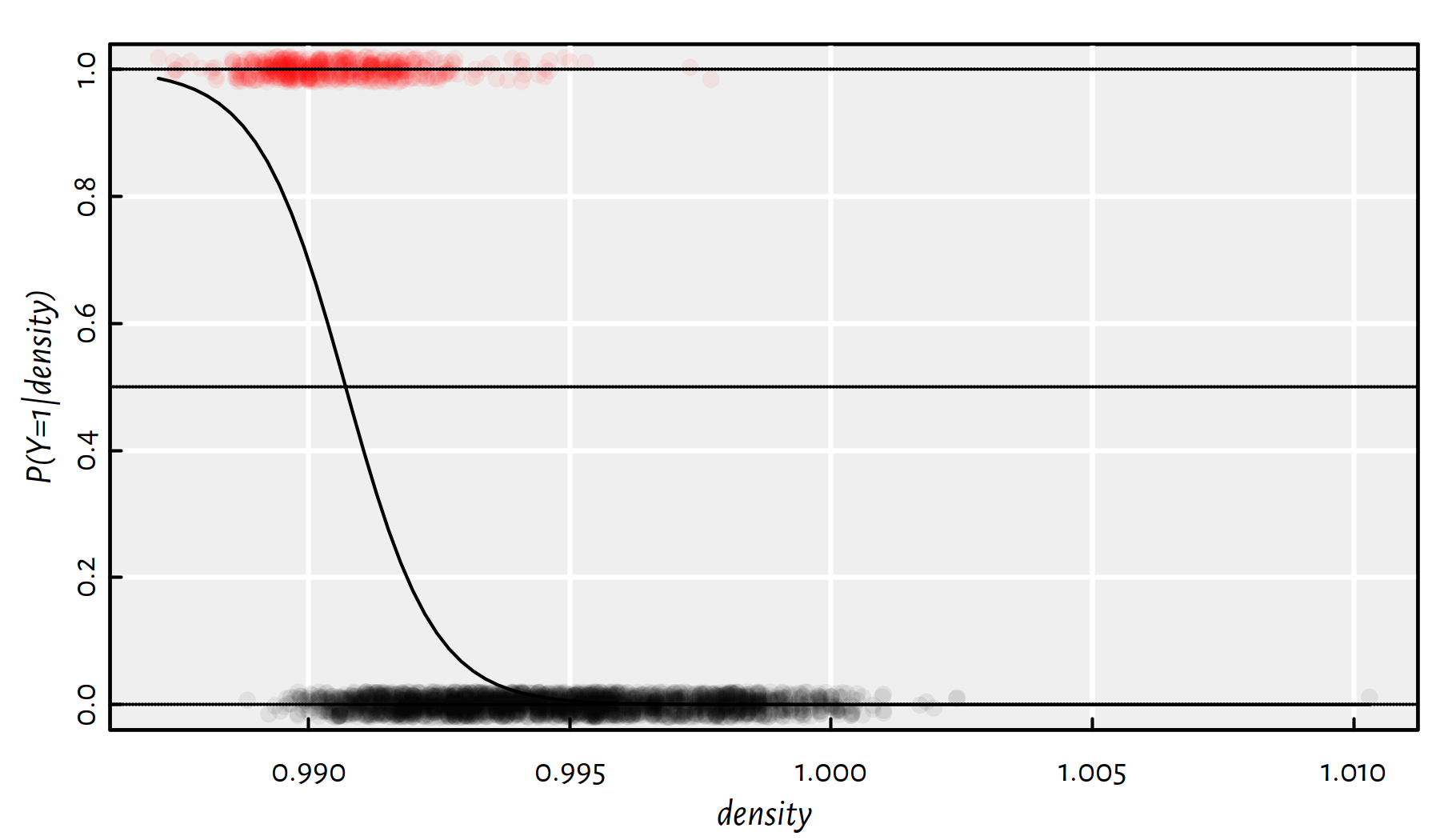Figure 4.10: The probability that a given wine is a high-alcohol one given its density; black and red points denote the actual observed data points from the class 0 and 1, respectively

Some predicted probabilities:

round(head(predict(f, XY_test, type="response"), 12), 2)
## 1602 1605 1607 1608 1609 1613 1614 1615 1621 1622 1623 1627
## 0.01 0.01 0.00 0.02 0.03 0.36 0.00 0.31 0.36 0.06 0.03 0.00

We classify $$Y$$ as 1 if the corresponding membership probability is greater than $$0.5$$.

Y_pred <- as.numeric(predict(f, XY_test, type="response")>0.5)
get_metrics(Y_pred, Y_test)
##        Acc       Prec        Rec          F         TN         FN
##    0.89796    0.72763    0.58991    0.65157 1573.00000  130.00000
##         FP         TP
##   70.00000  187.00000

And now a fit based on some other input variables:

(f <- glm(Y~density+residual.sugar+total.sulfur.dioxide,
data=XY_train, family=binomial("logit")))
##
## Call:  glm(formula = Y ~ density + residual.sugar + total.sulfur.dioxide,
##     family = binomial("logit"), data = XY_train)
##
## Coefficients:
##          (Intercept)               density        residual.sugar
##             2.50e+03             -2.53e+03              8.58e-01
## total.sulfur.dioxide
##             9.74e-03
##
## Degrees of Freedom: 2937 Total (i.e. Null);  2934 Residual
## Null Deviance:       2670
## Residual Deviance: 920   AIC: 928
Y_pred <- as.numeric(predict(f, XY_test, type="response")>0.5)
get_metrics(Y_pred, Y_test)
##        Acc       Prec        Rec          F         TN         FN
##    0.93214    0.82394    0.73817    0.77870 1593.00000   83.00000
##         FP         TP
##   50.00000  234.00000
Exercise.

Try fitting different models based on other sets of features.

### 4.3.4 Loss Function: Cross-entropy

The fitting of the model can be written as an optimisation task:

$\min_{\beta_0, \beta_1,\dots, \beta_p\in\mathbb{R}} \frac{1}{n} \sum_{i=1}^n \epsilon\left(\hat{y}_i, y_i \right)$

where $$\epsilon(\hat{y}_i, y_i)$$ denotes the penalty that measures the “difference” between the true $$y_i$$ and its predicted version $$\hat{y}_i=\Pr(Y=1|\mathbf{x}_{i,\cdot},\boldsymbol\beta)$$.

In the ordinary regression, we used the squared residual $$\epsilon(\hat{y}_i, y_i) = (\hat{y}_i-y_i)^2$$. In logistic regression (the kind of a classifier we are interested in right now), we use the cross-entropy (a.k.a. log-loss, binary cross-entropy),

$\epsilon(\hat{y}_i,y_i) = - \left(y_i \log \hat{y}_i + (1-y_i) \log(1-\hat{y}_i)\right)$

The corresponding loss function has not only many nice statistical properties (** related to maximum likelihood estimation etc.) but also an intuitive interpretation.

Note that the predicted $$\hat{y}_i$$ is in $$(0,1)$$ and the true $$y_i$$ equals to either 0 or 1. Recall also that $$\log t\in(-\infty, 0)$$ for $$t\in (0,1)$$. Therefore, the formula for $$\epsilon(\hat{y}_i,y_i)$$ has a very intuitive behaviour:

• if true $$y_i=1$$, then the penalty becomes $$\epsilon(\hat{y}_i, 1) = -\log(\hat{y}_i)$$

• $$\hat{y}_i$$ is the probability that the classified input is indeed from class $$1$$
• we’d be happy if the classifier outputted $$\hat{y}_i\simeq 1$$ in this case; this is not penalised as $$-\log(t)\to 0$$ as $$t\to 1$$
• however, if the classifier is totally wrong, i.e., it thinks that $$\hat{y}_i\simeq 0$$, then the penalty will be very high, as $$-\log(t)\to+\infty$$ as $$t\to 0$$
• if true $$y_i=0$$, then the penalty becomes $$\epsilon(\hat{y}_i, 0) = -\log(1-\hat{y}_i)$$

• $$1-\hat{y}_i$$ is the predicted probability that the input is from class $$0$$
• we penalise heavily the case where $$1-\hat{y}_i$$ is small (we’d be happy if the classifier was sure that $$1-\hat{y}_i\simeq 1$$, because this is the ground-truth)

(*) Having said that, let’s expand the above formulae. The task of minimising cross-entropy in the binary logistic regression can be written as $$\min_{\boldsymbol\beta\in\mathbb{R}^{p+1}} E(\boldsymbol\beta)$$ with:

$E(\boldsymbol\beta)= -\frac{1}{n} \sum_{i=1}^n y_i \log \Pr(Y=1|\mathbf{x}_{i,\cdot},\boldsymbol\beta) + (1-y_i) \log(1-\Pr(Y=1|\mathbf{x}_{i,\cdot},\boldsymbol\beta))$

Taking into account that:

$\Pr(Y=1|\mathbf{x}_{i,\cdot},\boldsymbol\beta)= \frac{1}{1+e^{-(\beta_0 + \beta_1 x_{i,1}+\dots+\beta_p x_{i,p})}},$

we get:

$E(\boldsymbol\beta)= -\frac{1}{n} \sum_{i=1}^n \left( \begin{array}{r} y_i \log \displaystyle\frac{1}{1+e^{-(\beta_0 + \beta_1 x_{i,1}+\dots+\beta_p x_{i,p})}}\\ + (1-y_i) \log \displaystyle\frac{e^{-(\beta_0 + \beta_1 x_{i,1}+\dots+\beta_p x_{i,p})}}{1+e^{-(\beta_0 + \beta_1 x_{i,1}+\dots+\beta_p x_{i,p})}} \end{array} \right).$

Logarithms are really practitioner-friendly functions, it holds:

• $$\log 1=0$$,
• $$\log e=1$$ (where $$e \simeq 2.71828$$ is the Euler constant; note that by writing $$\log$$ we mean the natural a.k.a. base-$$e$$ logarithm),
• $$\log xy = \log x + \log y$$,
• $$\log x^p = p\log x$$ (this is $$\log (x^p)$$, not $$(\log x)^p$$).

These facts imply, amongst others that:

• $$\log e^x = x \log e = x$$,
• $$\log \frac{x}{y} = \log x y^{-1} = \log x+\log y^{-1} = \log x - \log y$$ (of course for $$y\neq 0$$),
• $$\log \frac{1}{y} = -\log y$$

and so forth. Therefore, based on the fact that $$1/(1+e^{-x})=e^x/(1+e^x)$$, the above optimisation problem can be rewritten as:

$E(\boldsymbol\beta)= \frac{1}{n} \sum_{i=1}^n \left( \begin{array}{r} y_i \log \left(1+e^{-(\beta_0 + \beta_1 x_{i,1}+\dots+\beta_p x_{i,p})}\right)\\ + (1-y_i) \log \left(1+e^{+(\beta_0 + \beta_1 x_{i,1}+\dots+\beta_p x_{i,p})}\right) \end{array} \right)$

or, if someone prefers:

$E(\boldsymbol\beta)= \frac{1}{n} \sum_{i=1}^n \left( (1-y_i)\left(\beta_0 + \beta_1 x_{i,1}+\dots+\beta_p x_{i,p}\right) +\log \left(1+e^{-(\beta_0 + \beta_1 x_{i,1}+\dots+\beta_p x_{i,p})}\right) \right).$

It turns out that there is no analytical formula for the optimal set of parameters ($$\beta_0,\beta_1,\dots,\beta_p$$ minimising the log-loss). In the chapter on optimisation, we shall see that the solution to the logistic regression can be solved numerically by means of quite simple iterative algorithms. The two expanded formulae have lost the appealing interpretation of the original one, however, it’s more numerically well-behaving, see, e.g., the log1p() function in base R or, even better, fermi_dirac_0() in the gsl package.

## 4.4 Exercises in R

### 4.4.1 EdStats – Preparing Data

In this exercise, we will prepare the EdStats dataset for further analysis. The file edstats_2019.csv provides us with many country-level Education Statistics extracted from the World Bank’s Databank, see https://databank.worldbank.org/. Databank aggregates information from such sources as the UNESCO Institute for Statistics, OECD Programme for International Student Assessment (PISA) etc. The official description reads:

“The World Bank EdStats Query holds around 2,500 internationally comparable education indicators for access, progression, completion, literacy, teachers, population, and expenditures. The indicators cover the education cycle from pre-primary to tertiary education. The query also holds learning outcome data from international learning assessments (PISA, TIMSS, etc.), equity data from household surveys, and projection data to 2050.”

edstats_2019.csv was compiled on 24 April 2020 and lists indicators reported between 2010 and 2019. First, let’s load the dataset:

edstats_2019 <- read.csv("datasets/edstats_2019.csv",
comment.char="#")
head(edstats_2019)
##   CountryName CountryCode
## 1 Afghanistan         AFG
## 2 Afghanistan         AFG
## 3 Afghanistan         AFG
## 4 Afghanistan         AFG
## 5 Afghanistan         AFG
## 6 Afghanistan         AFG
##                                                    Series
## 1     Government expenditure on education as % of GDP (%)
## 2              Gross enrolment ratio, primary, female (%)
## 3                 Net enrolment rate, primary, female (%)
## 4                 Primary completion rate, both sexes (%)
## 5         PISA: Mean performance on the mathematics scale
## 6 PISA: Mean performance on the mathematics scale. Female
##                Code   Y2010  Y2011   Y2012   Y2013   Y2014   Y2015
## 1 SE.XPD.TOTL.GD.ZS  3.4794  3.462  2.6042  3.4545  3.6952  3.2558
## 2    SE.PRM.ENRR.FE 80.6355 80.937 86.3288 85.9021 86.7296 83.5044
## 3    SE.PRM.NENR.FE      NA     NA      NA      NA      NA      NA
## 4    SE.PRM.CMPT.ZS      NA     NA      NA      NA      NA      NA
## 5       LO.PISA.MAT      NA     NA      NA      NA      NA      NA
## 6    LO.PISA.MAT.FE      NA     NA      NA      NA      NA      NA
##     Y2016   Y2017  Y2018 Y2019
## 1  4.2284  4.0589     NA    NA
## 2 82.5584 82.0803 82.850    NA
## 3      NA      NA     NA    NA
## 4 79.9346 84.4150 85.625    NA
## 5      NA      NA     NA    NA
## 6      NA      NA     NA    NA

This data frame is in a “long” format, where each indicator for each country is given in its own row. Note that some indicators are not surveyed/updated every year.

Exercise.

Convert edstats_2019 to a “wide” format (one row per country, each indicator in its own column) based on the most recent observed indicators.

First we need a function that returns the last non-missing value in a given numeric vector. To recall, na.omit(), removes all missing values and tail() can be used to access the last observation easily. Unfortunately, if the vector is consists of missing values only, the removal of NAs leads to an empty sequence. However, the trick we can use is that by extracting the first element from an empty vector by using [...], we get a NA.

last_non_na <- function(x) tail(na.omit(x), 1)
last_non_na(c(1, 2, NA, 3,NA,NA)) # example 1
##  3
last_non_na(c(NA,NA,NA,NA,NA,NA)) # example 2
##  NA

Let’s extract the most recent indicator from each row in edstats_2019.

values <- apply(edstats_2019[-(1:4)], 1, last_non_na)
head(values)
##   4.0589 82.8503      NA 85.6253      NA      NA

Now, we shall create a data frame with 3 columns: name of the country, indicator code, indicator value. Let’s order it with respect to the first two columns.

edstats_2019 <- edstats_2019[c("CountryName", "Code")]
# add a new column at the righthand end:
edstats_2019["Value"] <- values
edstats_2019 <- edstats_2019[
order(edstats_2019$CountryName, edstats_2019$Code), ]
head(edstats_2019)
##    CountryName           Code  Value
## 59 Afghanistan    HD.HCI.AMRT 0.7797
## 57 Afghanistan HD.HCI.AMRT.FE 0.8018
## 58 Afghanistan HD.HCI.AMRT.MA 0.7597
## 53 Afghanistan    HD.HCI.EYRS 8.5800
## 51 Afghanistan HD.HCI.EYRS.FE 6.7300
## 52 Afghanistan HD.HCI.EYRS.MA 9.2100

To convert the data frame to a “wide” format, many readers would choose the pivot_wider() function from the tidyr package (amongst others).

library("tidyr")
edstats <- as.data.frame(
pivot_wider(edstats_2019, names_from="Code", values_from="Value")
)
edstats[1, 1:7]
##   CountryName HD.HCI.AMRT HD.HCI.AMRT.FE HD.HCI.AMRT.MA HD.HCI.EYRS
## 1 Afghanistan      0.7797         0.8018         0.7597        8.58
##   HD.HCI.EYRS.FE HD.HCI.EYRS.MA
## 1           6.73           9.21

On a side note (*), the above solution is of course perfectly fine and we can now live long and prosper. Nevertheless, we are here to learn new skills, so let’s note that it has the drawback that it required us to search for the answer on the internet (and go through many “answers” that actually don’t work). If we are not converting between the long and the wide formats on a daily basis, this might not be worth the hassle (moreover, there’s no guarantee that this function will work the same way in the future, that the package we relied on will provide the same API etc.).

Instead, by relaying on a bit deeper knowledge of R programming (which we already have, see Appendices A-D of our book), we could implement the relevant procedure manually. The downside is that this requires us to get out of our comfort zone and… think.

First, let’s generate the list of all countries and indicators:

countries  <- unique(edstats_2019$CountryName) head(countries) ##  "Afghanistan" "Albania" "Algeria" "American Samoa" ##  "Andorra" "Angola" indicators <- unique(edstats_2019$Code)
head(indicators)
##  "HD.HCI.AMRT"    "HD.HCI.AMRT.FE" "HD.HCI.AMRT.MA" "HD.HCI.EYRS"
##  "HD.HCI.EYRS.FE" "HD.HCI.EYRS.MA"

Second, note that edstats_2019 gives all the possible combinations (pairs) of the indexes and countries:

nrow(edstats_2019) # number of rows in edstats_2019
##  23852
length(countries)*length(indicators) # number of pairs
##  23852

Looking at the numbers in the Value column of edstats_2019, this will exactly provide us with our desired “wide” data matrix, if we read it in a rowwise manner. Hence, we can use matrix(..., byrow=TRUE) to generate it:

# edstats_2019 is already sorted w.r.t. CountryName and Code
edstats2 <- cbind(
CountryName=countries, # first column
as.data.frame(
matrix(edstats_2019$Value, byrow=TRUE, ncol=length(indicators), dimnames=list(NULL, indicators) ))) identical(edstats, edstats2) ##  TRUE Exercise. Export edstats to a CSV file. Click here for a solution. This can be done as follows: write.csv(edstats, "edstats_2019_wide.csv", row.names=FALSE) We didn’t export the row names, because they’re useless in our case. Exercise. Explore edstats_meta.csv to understand the meaning of the EdStats indicators. Click here for a solution. First, let’s load the dataset: meta <- read.csv("datasets/edstats_meta.csv") names(meta) # column names ##  "Code" "Series" "Definition" "Source" "Topic" The Series column deciphers each indicator’s meaning. For instance, LO.PISA.MAT gives: meta[meta$Code=="LO.PISA.MAT", "Series"]
##  "PISA: Mean performance on the mathematics scale"

To get more information, we can take a look at the Definition column:

meta[meta$Code=="LO.PISA.MAT", "Definition"] which reads: Average score of 15-year-old students on the PISA mathematics scale. The metric for the overall mathematics scale is based on a mean for OECD countries of 500 points and a standard deviation of 100 points. Data reflects country performance in the stated year according to PISA reports, but may not be comparable across years or countries. Consult the PISA website for more detailed information: http://www.oecd.org/pisa/. ### 4.4.2 EdStats – Where Girls Are Better at Maths Than Boys? In this task we will consider the “wide” version of the EdStats dataset: edstats <- read.csv("datasets/edstats_2019_wide.csv", comment.char="#") edstats[1, 1:6] ## CountryName HD.HCI.AMRT HD.HCI.AMRT.FE HD.HCI.AMRT.MA HD.HCI.EYRS ## 1 Afghanistan 0.7797 0.8018 0.7597 8.58 ## HD.HCI.EYRS.FE ## 1 6.73 meta <- read.csv("datasets/edstats_meta.csv") This dataset is small, moreover, we’ll be more interested in the description (understanding) of data, not prediction of the response variable to unobserved samples. Note that we have the population of the World countries at hand here (new countries do not arise on a daily basis). Therefore, a train-test split won’t be performed. Exercise. Add a 0/1 factor-type variable girls_rule_maths that is equal to 1 if and only if a country’s average score of 15-year-old female students on the PISA mathematics scale is greater than the corresponding indicator for the male ones. Click here for a solution. Recall that a conversion of a logical value to a number yields 1 for TRUE and 0 for FALSE. Hence: edstats$girls_rule_maths <-
factor(as.numeric(
edstats$LO.PISA.MAT.FE>edstats$LO.PISA.MAT.MA
))
head(edstats$girls_rule_maths, 10) ##  <NA> 1 1 <NA> <NA> <NA> <NA> <NA> 0 <NA> ## Levels: 0 1 Unfortunately, there are many missing values in the dataset. More precisely: sum(is.na(edstats$girls_rule_maths)) # count
##  187
mean(is.na(edstats$girls_rule_maths)) # proportion ##  0.69776 Countries such as Egypt, India, Iran or Venezuela are not amongst the 79 members of the Programme for International Student Assessment. Thus, we’ll have to deal with the data we have. The percentage of counties where “girls rule” is equal to: mean(edstats$girls_rule_maths==1, na.rm=TRUE)
##  0.33333

Here is the list of those counties:

as.character(na.omit(
edstats[edstats$girls_rule_maths==1, "CountryName"] )) ##  "Albania" "Algeria" ##  "Brunei Darussalam" "Bulgaria" ##  "Cyprus" "Dominican Republic" ##  "Finland" "Georgia" ##  "Hong Kong SAR, China" "Iceland" ##  "Indonesia" "Israel" ##  "Jordan" "Lithuania" ##  "Malaysia" "Malta" ##  "Moldova" "North Macedonia" ##  "Norway" "Philippines" ##  "Qatar" "Saudi Arabia" ##  "Sweden" "Thailand" ##  "Trinidad and Tobago" "United Arab Emirates" ##  "Vietnam" Exercise. Learn a decision tree that distinguishes between the countries where girls are better at maths than boys and assess the quality of this classifier. Click here for a solution. Let’s first create a subset of edstats that doesn’t include the country names as well as the boys’ and girls’ math scores. edstats_subset <- edstats[!(names(edstats) %in% c("CountryName", "LO.PISA.MAT.FE", "LO.PISA.MAT.MA"))] Fitting and plotting (see Figure 4.11) of the tree can be performed as follows: library("rpart") library("rpart.plot") tree <- rpart(girls_rule_maths~., data=edstats_subset, method="class", model=TRUE) rpart.plot(tree)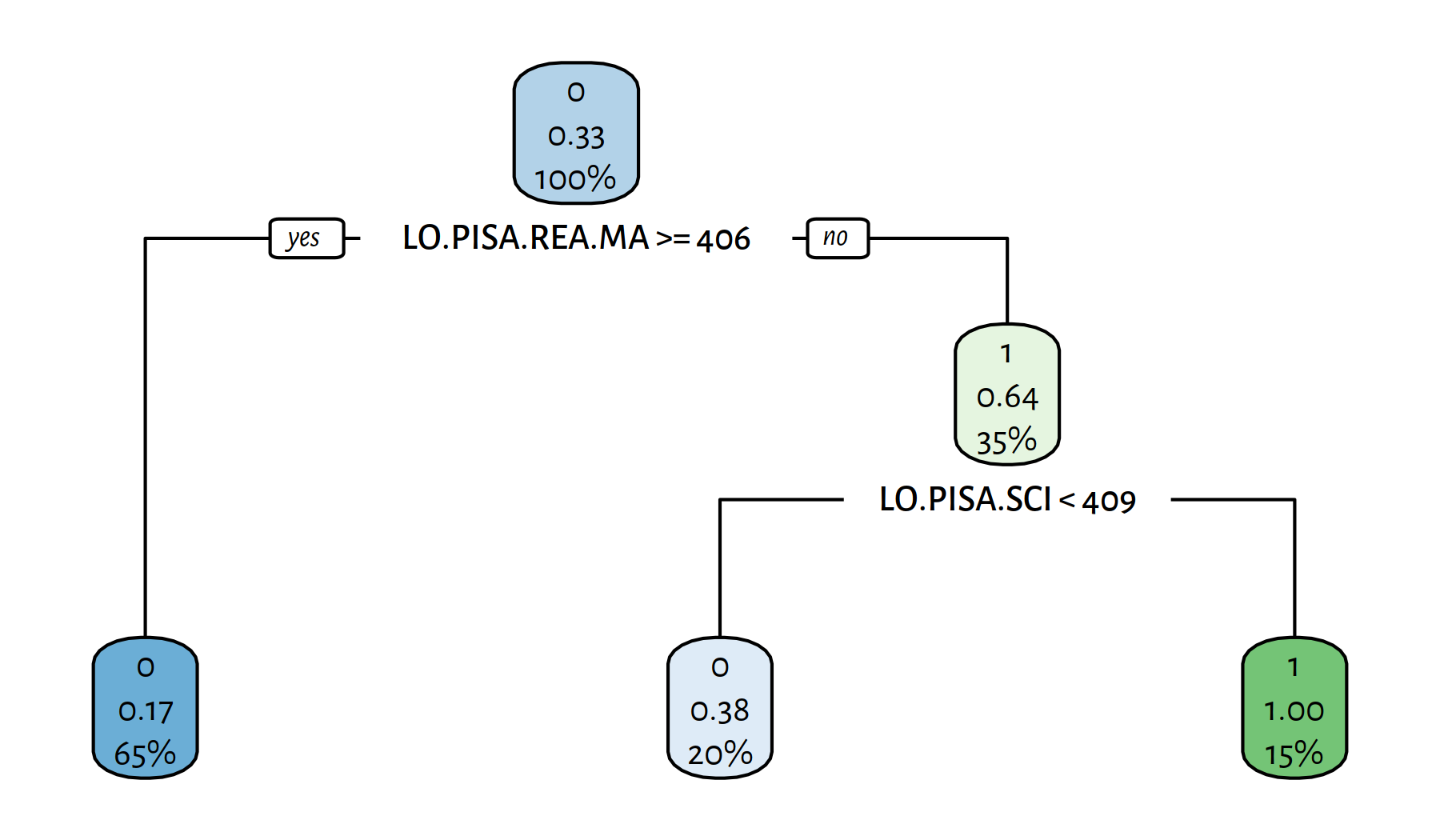Figure 4.11: A decision tree explaining the girls_rule_maths variable The variables included in the model are: Note that the decision rules are well-interpretable, we can make a whole story around it. Whether or not it is actually true – is a different… story. To compute the basic classifier performance scores, let’s recall the get_metrics() function: get_metrics <- function(Y_pred, Y_test) { C <- table(Y_pred, Y_test) # confusion matrix stopifnot(dim(C) == c(2, 2)) c(Acc=(C[1,1]+C[2,2])/sum(C), # accuracy Prec=C[2,2]/(C[2,2]+C[2,1]), # precision Rec=C[2,2]/(C[2,2]+C[1,2]), # recall F=C[2,2]/(C[2,2]+0.5*C[1,2]+0.5*C[2,1]), # F-measure # Confusion matrix items: TN=C[1,1], FN=C[1,2], FP=C[2,1], TP=C[2,2] ) # return a named vector } Now we can judge the tree’s character: Y_pred <- predict(tree, edstats_subset, type="class") get_metrics(Y_pred, edstats_subset$girls_rule_maths)
##      Acc     Prec      Rec        F       TN       FN       FP       TP
##  0.81481  1.00000  0.44444  0.61538 54.00000 15.00000  0.00000 12.00000
Exercise.

Learn a decision tree that this time doesn’t rely on any of the PISA indicators.

Let’s remove the unwanted variables:

edstats_subset <- edstats[!(names(edstats) %in%
c("LO.PISA.MAT", "LO.PISA.MAT.FE", "LO.PISA.MAT.MA",
"LO.PISA.REA", "LO.PISA.REA.FE", "LO.PISA.REA.MA",
"LO.PISA.SCI", "LO.PISA.SCI.FE", "LO.PISA.SCI.MA",
"CountryName"))]

On a side note, this could be done more easily by calling, e.g., stri_startswith_fixed(names(edstats), "LO.PISA") from the stringi package.

Fitting and plotting (see Figure 4.12) of the tree:

tree <- rpart(girls_rule_maths~., data=edstats_subset,
method="class", model=TRUE)
rpart.plot(tree)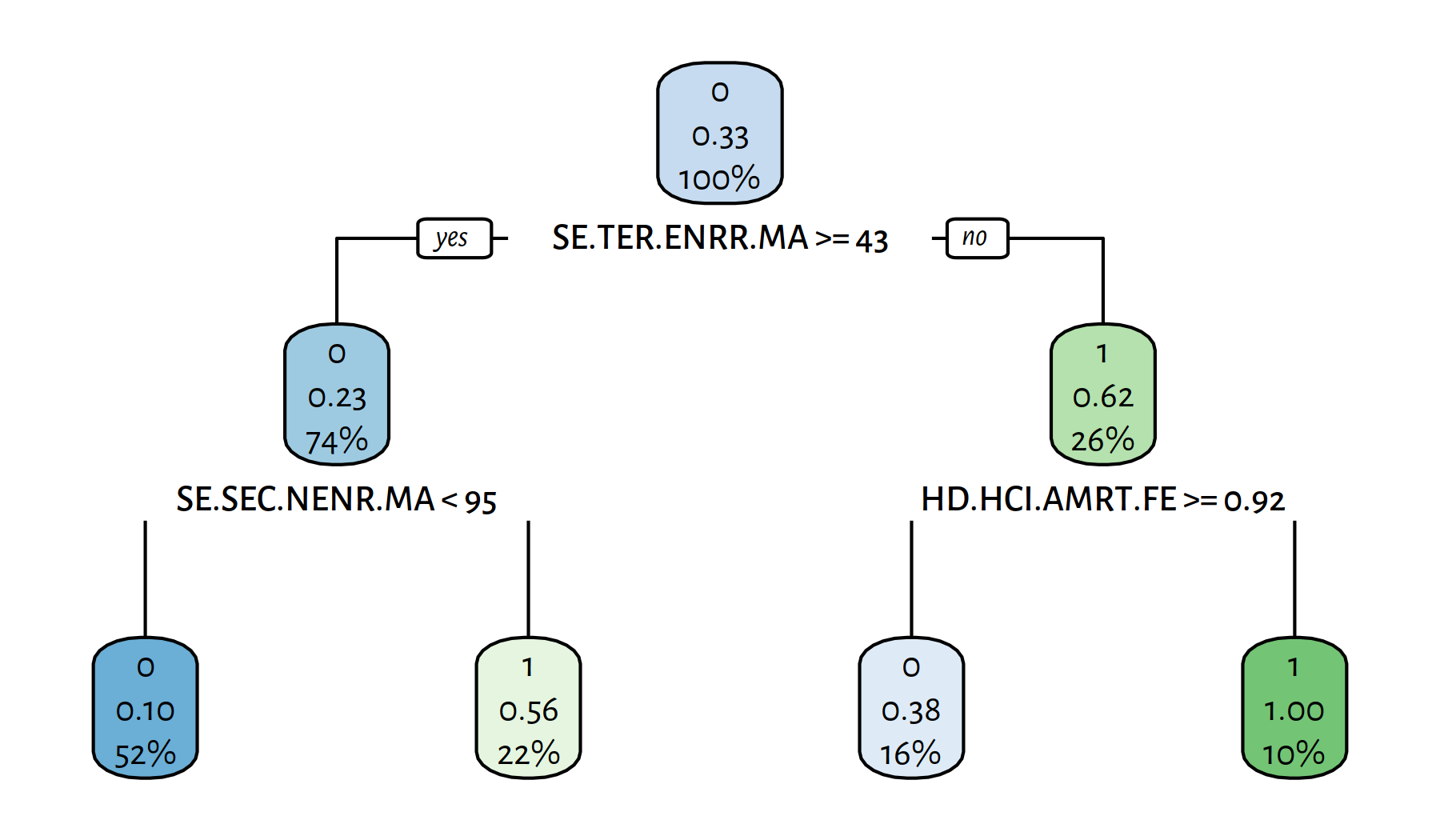Figure 4.12: Another decision tree explaining the girls_rule_maths variable

Performance metrics:

Y_pred <- predict(tree, edstats, type="class")
get_metrics(Y_pred, edstats_subset$girls_rule_maths) ## Acc Prec Rec F TN FN FP TP ## 0.79012 0.69231 0.66667 0.67925 46.00000 9.00000 8.00000 18.00000 It’s interesting to note that some of the goodness-of-fit measures are actually higher now. The variables included in the model are: ### 4.4.3 EdStats and World Factbook – Joining Forces In the course of our data science journey, we have considered two datasets dealing with country-level indicators: the World Factbook and World Bank’s EdStats. factbook <- read.csv("datasets/world_factbook_2020.csv", comment.char="#") edstats <- read.csv("datasets/edstats_2019_wide.csv", comment.char="#") Let’s combine the information they provide and see if we come up with a better model of where girls’ math scores are higher. Exercise. Some country names in one dataset don’t match those in the other one, for instance: Czech Republic vs. Czechia, Myanmar vs. Burma, etc. Resolve these conflicts as best you can. Click here for a solution. To get a list of the mismatched country names, we can call either: factbook$country[!(factbook$country %in% edstats$CountryName)]

or:

edstats$CountryName[!(edstats$CountryName %in% factbook$country)] Unfortunately, the data need to be cleaned manually – it’s a tedious task. The following consists of what we hope are the best matches between the two datasets (yet, the list is not perfect; in particular, the Republic of North Macedonia is completely missing in one of the datasets): from_to <- matrix(ncol=2, byrow=TRUE, c( # FROM (edstats) # TO (factbook) "Brunei Darussalam" , "Brunei" , "Congo, Dem. Rep." , "Congo, Democratic Republic of the" , "Congo, Rep." , "Congo, Republic of the" , "Czech Republic" , "Czechia" , "Egypt, Arab Rep." , "Egypt" , "Hong Kong SAR, China" , "Hong Kong" , "Iran, Islamic Rep." , "Iran" , "Korea, Dem. People’s Rep." , "Korea, North" , "Korea, Rep." , "Korea, South" , "Kyrgyz Republic" , "Kyrgyzstan" , "Lao PDR" , "Laos" , "Macao SAR, China" , "Macau" , "Micronesia, Fed. Sts." , "Micronesia, Federated States of" , "Myanmar" , "Burma" , "Russian Federation" , "Russia" , "Slovak Republic" , "Slovakia" , "St. Kitts and Nevis" , "Saint Kitts and Nevis" , "St. Lucia" , "Saint Lucia" , "St. Martin (French part)" , "Saint Martin" , "St. Vincent and the Grenadines", "Saint Vincent and the Grenadines" , "Syrian Arab Republic" , "Syria" , "Venezuela, RB" , "Venezuela" , "Virgin Islands (U.S.)" , "Virgin Islands" , "Yemen, Rep." , "Yemen" )) Conversion of the names: for (i in 1:nrow(from_to)) { edstats$CountryName[edstats$CountryName==from_to[i,1]] <- from_to[i,2] } On a side note (*), this could be done with a single call to a function in the stringi package: library("stringi") edstats$CountryName <- stri_replace_all_fixed(edstats$CountryName, from_to[,1], from_to[,2], vectorize_all=FALSE) Exercise. Merge (join) the two datasets based on the country names. Click here for a solution. This can be done by means of the merge() function. edbook <- merge(edstats, factbook, by.x="CountryName", by.y="country") ncol(edbook) # how many columns we have now ##  157 Exercise. Learn a decision tree that distinguishes between the countries where girls are better at maths than boys and assess the quality of this classifier. Click here for a solution. We proceed as in one of the previous exercises: edbook$girls_rule_maths <-
factor(as.numeric(
edbook$LO.PISA.MAT.FE>edbook$LO.PISA.MAT.MA
))
edbook_subset <- edbook[!(names(edbook) %in%
c("CountryName", "LO.PISA.MAT.FE", "LO.PISA.MAT.MA"))]

Fitting and plotting (see Figure 4.13):

library("rpart")
library("rpart.plot")
tree <- rpart(girls_rule_maths~., data=edbook_subset,
method="class", model=TRUE)
rpart.plot(tree)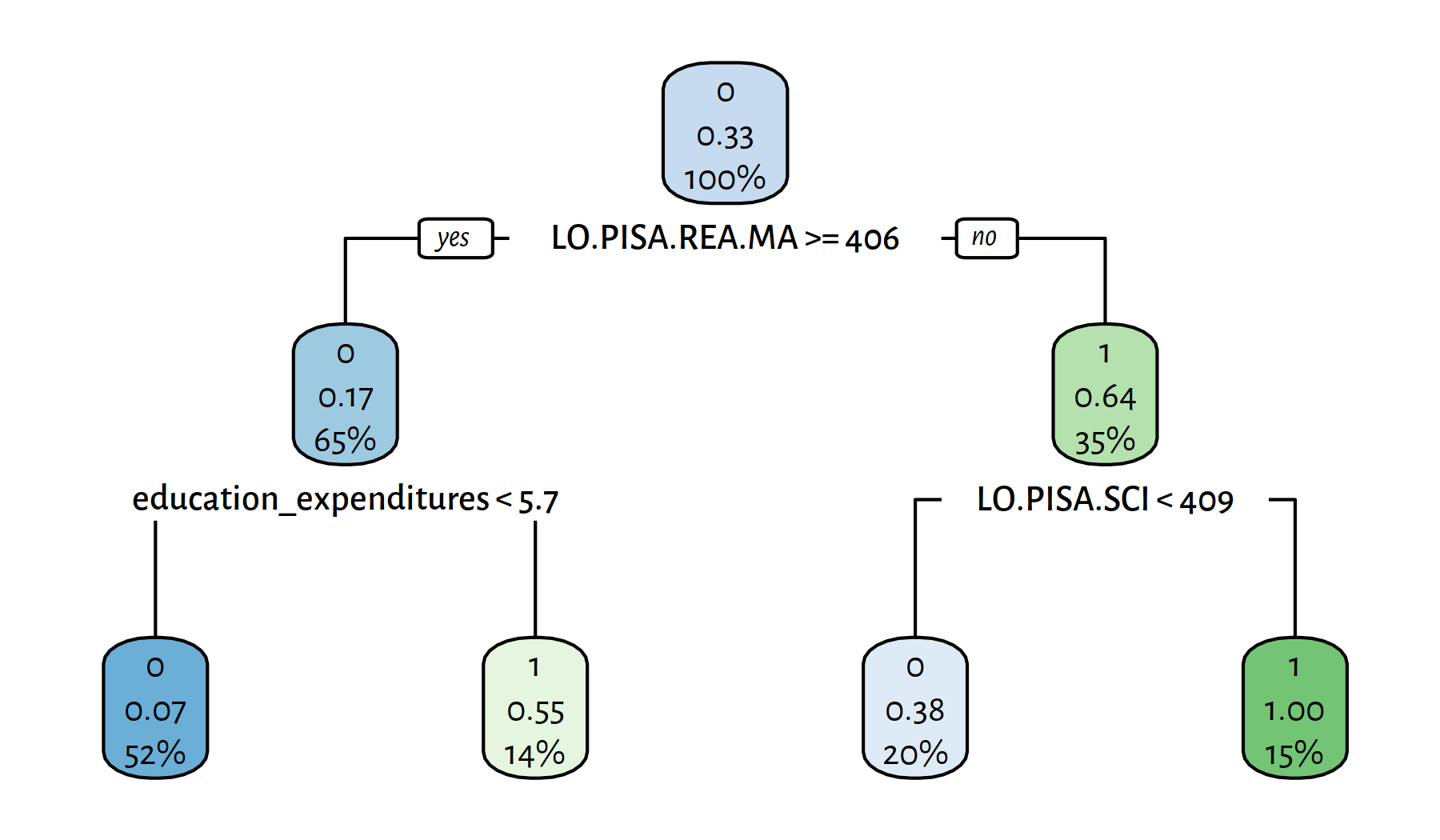Figure 4.13: Yet another decision tree explaining the girls_rule_maths variable

Performance metrics:

Y_pred <- predict(tree, edbook_subset, type="class")
get_metrics(Y_pred, edbook_subset$girls_rule_maths) ## Acc Prec Rec F TN FN FP TP ## 0.82716 0.78261 0.66667 0.72000 49.00000 9.00000 5.00000 18.00000 The variables included in the model are: This is… not at all enlightening. Rest assured that experts in education or econometrics for whom we work in this (imaginary) project would raise many questions at this very point. Merely applying some computational procedure on a dataset doesn’t cut it; it’s too early to ask for a paycheque. Classifiers are just blind tools in our gentle yet firm hands; new questions are risen, new answers must be sought. Further explorations are of course left as an exercise to the kind reader. ### 4.4.4 EdStats – Fitting of Binary Logistic Regression Models In this task we’re going to consider the “wide” version of the EdStats dataset again: edstats <- read.csv("datasets/edstats_2019_wide.csv", comment.char="#") Let’s re-add the girls_rule_maths column just as in the previous exercise. Then, let’s create a subset of edstats that doesn’t include the country names as well as the boys’ and girls’ math scores. edstats$girls_rule_maths <-
factor(as.numeric(
edstats$LO.PISA.MAT.FE>edstats$LO.PISA.MAT.MA
))
edstats_subset <- edstats[!(names(edstats) %in%
c("CountryName", "LO.PISA.MAT.FE", "LO.PISA.MAT.MA"))]
Exercise.

Fit and assess a logistic regression model for girls_rule_maths as a function of LO.PISA.REA.MA+LO.PISA.SCI.

Fitting of the model:

(f1 <- glm(girls_rule_maths~LO.PISA.REA.MA+LO.PISA.SCI,
data=edstats_subset, family=binomial("logit")))
##
## Call:  glm(formula = girls_rule_maths ~ LO.PISA.REA.MA + LO.PISA.SCI,
##     family = binomial("logit"), data = edstats_subset)
##
## Coefficients:
##    (Intercept)  LO.PISA.REA.MA     LO.PISA.SCI
##         3.0927         -0.0882          0.0755
##
## Degrees of Freedom: 80 Total (i.e. Null);  78 Residual
##   (187 observations deleted due to missingness)
## Null Deviance:       103
## Residual Deviance: 77.9  AIC: 83.9

Performance metrics:

Y_pred <- as.numeric(predict(f1, edstats_subset, type="response")>0.5)
get_metrics(Y_pred, edstats_subset$girls_rule_maths) ## Acc Prec Rec F TN FN FP TP ## 0.79012 0.75000 0.55556 0.63830 49.00000 12.00000 5.00000 15.00000 Relate the above numbers to those reported for the fitted decision trees. Note that the fitted model is nicely interpretable: the lower the boys’ average result on the Reading Scale or the higher the country’s result on the Science Scale, the higher the probability for girls_rule_maths: example_X <- data.frame( LO.PISA.REA.MA=c(475, 450, 475, 500), LO.PISA.SCI= c(525, 525, 550, 500) ) cbind(example_X, Pr(Y=1)=predict(f1, example_X, type="response")) ## LO.PISA.REA.MA LO.PISA.SCI Pr(Y=1) ## 1 475 525 0.703342 ## 2 450 525 0.955526 ## 3 475 550 0.939986 ## 4 500 500 0.038094 Exercise. (*) Fit and assess a logistic regression model for girls_rule_maths featuring all LO.PISA.REA* and LO.PISA.SCI* as independent variables. Click here for a solution. Model fitting: (f2 <- glm(girls_rule_maths~LO.PISA.REA+LO.PISA.REA.FE+LO.PISA.REA.MA+ LO.PISA.SCI+LO.PISA.SCI.FE+LO.PISA.SCI.MA, data=edstats_subset, family=binomial("logit"))) ## Warning: glm.fit: fitted probabilities numerically 0 or 1 occurred ## ## Call: glm(formula = girls_rule_maths ~ LO.PISA.REA + LO.PISA.REA.FE + ## LO.PISA.REA.MA + LO.PISA.SCI + LO.PISA.SCI.FE + LO.PISA.SCI.MA, ## family = binomial("logit"), data = edstats_subset) ## ## Coefficients: ## (Intercept) LO.PISA.REA LO.PISA.REA.FE LO.PISA.REA.MA ## -2.265 1.268 -0.544 -0.734 ## LO.PISA.SCI LO.PISA.SCI.FE LO.PISA.SCI.MA ## 1.269 -0.157 -1.112 ## ## Degrees of Freedom: 80 Total (i.e. Null); 74 Residual ## (187 observations deleted due to missingness) ## Null Deviance: 103 ## Residual Deviance: 33 AIC: 47 The mysterious fitted probabilities numerically 0 or 1 occurred warning denotes convergence problems of the underlying optimisation (fitting) procedure: at least one of the model coefficients has had a fairly large order of magnitude and hence the fitted probabilities has come very close to 0 or 1. Recall that the probabilities are modelled by means of the logistic sigmoid function applied on the output of a linear combination of the dependent variables. Moreover, cross-entropy features a logarithm, and $$\log 0 = -\infty$$. This can be due to the fact that all the variables in the model are very correlated with each other (multicollinearity; an ill-conditioned problem). The obtained solution might be unstable – there might be many local optima and hence, different parameter vectors might be equally good. Moreover, it is likely that a small change in one of the inputs might lead to large change in the estimated model (* normally, we would attack this problem by employing some regularisation techniques). Of course, the model’s performance metrics can still be computed, but then it’s better if we treat it as a black box. Or, even better, reduce the number of independent variables and come up with a simpler model that serves its purpose better than this one. Y_pred <- as.numeric(predict(f2, edstats_subset, type="response")>0.5) get_metrics(Y_pred, edstats_subset$girls_rule_maths)
##      Acc     Prec      Rec        F       TN       FN       FP       TP
##  0.86420  0.83333  0.74074  0.78431 50.00000  7.00000  4.00000 20.00000

### 4.4.5 EdStats – Variable Selection in Binary Logistic Regression (*)

Back to our girls_rule_maths example, we still have so much to learn!

edstats <- read.csv("datasets/edstats_2019_wide.csv",
comment.char="#")
edstats$girls_rule_maths <- factor(as.numeric( edstats$LO.PISA.MAT.FE>edstats$LO.PISA.MAT.MA )) edstats_subset <- edstats[!(names(edstats) %in% c("CountryName", "LO.PISA.MAT.FE", "LO.PISA.MAT.MA"))] Exercise. Construct a binary logistic regression model via forward selection of variables. Click here for a solution. Just as in the linear regression case, we can rely on the step() function. model_empty <- girls_rule_maths~1 (model_full <- formula(model.frame(girls_rule_maths~., data=edstats_subset))) ## girls_rule_maths ~ HD.HCI.AMRT + HD.HCI.AMRT.FE + HD.HCI.AMRT.MA + ## HD.HCI.EYRS + HD.HCI.EYRS.FE + HD.HCI.EYRS.MA + HD.HCI.HLOS + ## HD.HCI.HLOS.FE + HD.HCI.HLOS.MA + HD.HCI.MORT + HD.HCI.MORT.FE + ## HD.HCI.MORT.MA + HD.HCI.OVRL + HD.HCI.OVRL.FE + HD.HCI.OVRL.MA + ## IT.CMP.PCMP.P2 + IT.NET.USER.P2 + LO.PISA.MAT + LO.PISA.REA + ## LO.PISA.REA.FE + LO.PISA.REA.MA + LO.PISA.SCI + LO.PISA.SCI.FE + ## LO.PISA.SCI.MA + NY.GDP.MKTP.CD + NY.GDP.PCAP.CD + NY.GDP.PCAP.PP.CD + ## NY.GNP.PCAP.CD + NY.GNP.PCAP.PP.CD + SE.COM.DURS + SE.PRM.CMPT.FE.ZS + ## SE.PRM.CMPT.MA.ZS + SE.PRM.CMPT.ZS + SE.PRM.ENRL.TC.ZS + ## SE.PRM.ENRR + SE.PRM.ENRR.FE + SE.PRM.ENRR.MA + SE.PRM.NENR + ## SE.PRM.NENR.FE + SE.PRM.NENR.MA + SE.PRM.PRIV.ZS + SE.SEC.ENRL.TC.ZS + ## SE.SEC.ENRR + SE.SEC.ENRR.FE + SE.SEC.ENRR.MA + SE.SEC.NENR + ## SE.SEC.NENR.MA + SE.SEC.PRIV.ZS + SE.TER.ENRR + SE.TER.ENRR.FE + ## SE.TER.ENRR.MA + SE.TER.PRIV.ZS + SE.XPD.TOTL.GD.ZS + SL.TLF.ADVN.FE.ZS + ## SL.TLF.ADVN.MA.ZS + SL.TLF.ADVN.ZS + SP.POP.TOTL + SP.POP.TOTL.FE.IN + ## SP.POP.TOTL.MA.IN + SP.PRM.TOTL.FE.IN + SP.PRM.TOTL.IN + ## SP.PRM.TOTL.MA.IN + SP.SEC.TOTL.FE.IN + SP.SEC.TOTL.IN + ## SP.SEC.TOTL.MA.IN + UIS.PTRHC.56 + UIS.SAP.CE + UIS.SAP.CE.F + ## UIS.SAP.CE.M + UIS.X.PPP.1.FSGOV + UIS.X.PPP.2T3.FSGOV + ## UIS.X.PPP.5T8.FSGOV + UIS.X.US.1.FSGOV + UIS.X.US.2T3.FSGOV + ## UIS.X.US.5T8.FSGOV + UIS.XGDP.1.FSGOV + UIS.XGDP.23.FSGOV + ## UIS.XGDP.56.FSGOV + UIS.XUNIT.GDPCAP.1.FSGOV + UIS.XUNIT.GDPCAP.23.FSGOV + ## UIS.XUNIT.GDPCAP.5T8.FSGOV + UIS.XUNIT.PPP.1.FSGOV.FFNTR + ## UIS.XUNIT.PPP.2T3.FSGOV.FFNTR + UIS.XUNIT.PPP.5T8.FSGOV.FFNTR + ## UIS.XUNIT.US.1.FSGOV.FFNTR + UIS.XUNIT.US.23.FSGOV.FFNTR + ## UIS.XUNIT.US.5T8.FSGOV.FFNTR f <- step(glm(model_empty, data=edstats_subset, family=binomial("logit")), scope=model_full, direction="forward") ## Start: AIC=105.12 ## girls_rule_maths ~ 1 ## Error in model.matrix.default(Terms, m, contrasts.arg = object$contrasts): variable 1 has no levels

Melbourne, we have a problem! Our dataset has too many missing values, and those cannot be present in a logistic regression model (it’s based on a linear combination of variables, and sums/products involving NAs yield NAs…).

Looking at the manual of ?step, we see that the default NA handling is via na.omit(), and that, when applied on a data frame, results in the removal of all the rows, where there is at least one NA. Sadly, it’s too invasive.

We should get rid of the data blanks manually. First, definitely, we should remove all the rows where girls_rule_maths is unknown:

edstats_subset <-
edstats_subset[!is.na(edstats_subset$girls_rule_maths),] We are about to apply the forward selection process, whose purpose is to choose variables for a model. Therefore, instead of removing any more rows, we should remove the… columns with missing data: edstats_subset <- edstats_subset[,colSums(sapply(edstats_subset, is.na))==0] (*) Alternatively, we could apply some techniques of missing data imputation; this is beyond the scope of this book. For instance, NAs could be replaced by the averages of their respective columns. We are ready now to make use of step(). model_empty <- girls_rule_maths~1 (model_full <- formula(model.frame(girls_rule_maths~., data=edstats_subset))) ## girls_rule_maths ~ IT.NET.USER.P2 + LO.PISA.MAT + LO.PISA.REA + ## LO.PISA.REA.FE + LO.PISA.REA.MA + LO.PISA.SCI + LO.PISA.SCI.FE + ## LO.PISA.SCI.MA + NY.GDP.MKTP.CD + NY.GDP.PCAP.CD + SP.POP.TOTL f <- step(glm(model_empty, data=edstats_subset, family=binomial("logit")), scope=model_full, direction="forward") ## Start: AIC=105.12 ## girls_rule_maths ~ 1 ## ## Df Deviance AIC ## + LO.PISA.REA.MA 1 90.9 94.9 ## + LO.PISA.SCI.MA 1 93.3 97.3 ## + NY.GDP.MKTP.CD 1 94.2 98.2 ## + LO.PISA.REA 1 95.0 99.0 ## + LO.PISA.SCI 1 96.9 100.9 ## + LO.PISA.MAT 1 97.2 101.2 ## + LO.PISA.REA.FE 1 97.9 101.9 ## + LO.PISA.SCI.FE 1 99.4 103.4 ## <none> 103.1 105.1 ## + SP.POP.TOTL 1 101.9 105.9 ## + NY.GDP.PCAP.CD 1 102.3 106.3 ## + IT.NET.USER.P2 1 103.1 107.1 ## ## Step: AIC=94.93 ## girls_rule_maths ~ LO.PISA.REA.MA ## ## Df Deviance AIC ## + LO.PISA.REA 1 42.8 48.8 ## + LO.PISA.REA.FE 1 50.5 56.5 ## + LO.PISA.SCI.FE 1 65.4 71.4 ## + LO.PISA.SCI 1 77.9 83.9 ## + LO.PISA.MAT 1 83.5 89.5 ## + NY.GDP.MKTP.CD 1 87.4 93.4 ## + IT.NET.USER.P2 1 87.5 93.5 ## <none> 90.9 94.9 ## + NY.GDP.PCAP.CD 1 89.2 95.2 ## + LO.PISA.SCI.MA 1 89.2 95.2 ## + SP.POP.TOTL 1 90.2 96.2 ## ## Step: AIC=48.83 ## girls_rule_maths ~ LO.PISA.REA.MA + LO.PISA.REA ## ## Df Deviance AIC ## <none> 42.8 48.8 ## + LO.PISA.SCI.FE 1 40.9 48.9 ## + SP.POP.TOTL 1 41.2 49.2 ## + NY.GDP.PCAP.CD 1 41.3 49.3 ## + LO.PISA.SCI 1 42.0 50.0 ## + LO.PISA.MAT 1 42.4 50.4 ## + IT.NET.USER.P2 1 42.7 50.7 ## + LO.PISA.SCI.MA 1 42.7 50.7 ## + NY.GDP.MKTP.CD 1 42.7 50.7 ## + LO.PISA.REA.FE 1 42.8 50.8 print(f) ## ## Call: glm(formula = girls_rule_maths ~ LO.PISA.REA.MA + LO.PISA.REA, ## family = binomial("logit"), data = edstats_subset) ## ## Coefficients: ## (Intercept) LO.PISA.REA.MA LO.PISA.REA ## -0.176 -0.600 0.577 ## ## Degrees of Freedom: 80 Total (i.e. Null); 78 Residual ## Null Deviance: 103 ## Residual Deviance: 42.8 AIC: 48.8 Y_pred <- as.numeric(predict(f, edstats_subset, type="response")>0.5) get_metrics(Y_pred, edstats_subset$girls_rule_maths)
##      Acc     Prec      Rec        F       TN       FN       FP       TP
##  0.88889  0.84615  0.81481  0.83019 50.00000  5.00000  4.00000 22.00000
Exercise.

Choose a model via backward elimination.

Having a dataset with missing values removed, this is easy now:

f <- suppressWarnings( # yeah, yeah, yeah...
# fitted probabilities numerically 0 or 1 occurred
step(glm(model_full, data=edstats_subset, family=binomial("logit")),
scope=model_empty, direction="backward")
)
## Start:  AIC=50.83
## girls_rule_maths ~ IT.NET.USER.P2 + LO.PISA.MAT + LO.PISA.REA +
##     LO.PISA.REA.FE + LO.PISA.REA.MA + LO.PISA.SCI + LO.PISA.SCI.FE +
##     LO.PISA.SCI.MA + NY.GDP.MKTP.CD + NY.GDP.PCAP.CD + SP.POP.TOTL
##
##                  Df Deviance  AIC
## - LO.PISA.MAT     1     26.8 48.8
## - LO.PISA.SCI.MA  1     26.8 48.8
## - NY.GDP.PCAP.CD  1     26.9 48.9
## - LO.PISA.SCI     1     26.9 48.9
## - LO.PISA.SCI.FE  1     27.1 49.1
## - LO.PISA.REA.FE  1     27.4 49.4
## - LO.PISA.REA     1     27.5 49.5
## - LO.PISA.REA.MA  1     27.6 49.6
## <none>                  26.8 50.8
## - IT.NET.USER.P2  1     29.3 51.3
## - NY.GDP.MKTP.CD  1     29.9 51.9
## - SP.POP.TOTL     1     31.7 53.7
##
## Step:  AIC=48.84
## girls_rule_maths ~ IT.NET.USER.P2 + LO.PISA.REA + LO.PISA.REA.FE +
##     LO.PISA.REA.MA + LO.PISA.SCI + LO.PISA.SCI.FE + LO.PISA.SCI.MA +
##     NY.GDP.MKTP.CD + NY.GDP.PCAP.CD + SP.POP.TOTL
##
##                  Df Deviance  AIC
## - LO.PISA.SCI.MA  1     26.8 46.8
## - NY.GDP.PCAP.CD  1     26.9 46.9
## - LO.PISA.SCI     1     27.0 47.0
## - LO.PISA.SCI.FE  1     27.1 47.1
## - LO.PISA.REA.FE  1     27.4 47.4
## - LO.PISA.REA     1     27.5 47.5
## - LO.PISA.REA.MA  1     27.6 47.6
## <none>                  26.8 48.8
## - IT.NET.USER.P2  1     29.3 49.3
## - NY.GDP.MKTP.CD  1     29.9 49.9
## - SP.POP.TOTL     1     31.7 51.7
##
## Step:  AIC=46.84
## girls_rule_maths ~ IT.NET.USER.P2 + LO.PISA.REA + LO.PISA.REA.FE +
##     LO.PISA.REA.MA + LO.PISA.SCI + LO.PISA.SCI.FE + NY.GDP.MKTP.CD +
##     NY.GDP.PCAP.CD + SP.POP.TOTL
##
##                  Df Deviance  AIC
## - NY.GDP.PCAP.CD  1     26.9 44.9
## <none>                  26.8 46.8
## - IT.NET.USER.P2  1     29.3 47.3
## - NY.GDP.MKTP.CD  1     29.9 47.9
## - LO.PISA.REA.FE  1     31.0 49.0
## - SP.POP.TOTL     1     31.8 49.8
## - LO.PISA.SCI     1     35.6 53.6
## - LO.PISA.SCI.FE  1     36.1 54.1
## - LO.PISA.REA     1     37.5 55.5
## - LO.PISA.REA.MA  1     50.9 68.9
##
## Step:  AIC=44.87
## girls_rule_maths ~ IT.NET.USER.P2 + LO.PISA.REA + LO.PISA.REA.FE +
##     LO.PISA.REA.MA + LO.PISA.SCI + LO.PISA.SCI.FE + NY.GDP.MKTP.CD +
##     SP.POP.TOTL
##
##                  Df Deviance  AIC
## <none>                  26.9 44.9
## - NY.GDP.MKTP.CD  1     30.5 46.5
## - IT.NET.USER.P2  1     31.0 47.0
## - LO.PISA.REA.FE  1     31.1 47.1
## - SP.POP.TOTL     1     33.0 49.0
## - LO.PISA.SCI     1     35.9 51.9
## - LO.PISA.SCI.FE  1     36.4 52.4
## - LO.PISA.REA     1     37.5 53.5
## - LO.PISA.REA.MA  1     50.9 66.9

The obtained model and its quality metrics:

print(f)
##
## Call:  glm(formula = girls_rule_maths ~ IT.NET.USER.P2 + LO.PISA.REA +
##     LO.PISA.REA.FE + LO.PISA.REA.MA + LO.PISA.SCI + LO.PISA.SCI.FE +
##     NY.GDP.MKTP.CD + SP.POP.TOTL, family = binomial("logit"),
##     data = edstats_subset)
##
## Coefficients:
##    (Intercept)  IT.NET.USER.P2     LO.PISA.REA  LO.PISA.REA.FE
##      -1.66e+01        1.61e-01        1.85e+00       -8.00e-01
## LO.PISA.REA.MA     LO.PISA.SCI  LO.PISA.SCI.FE  NY.GDP.MKTP.CD
##      -1.03e+00       -1.35e+00        1.32e+00       -4.95e-12
##    SP.POP.TOTL
##       6.20e-08
##
## Degrees of Freedom: 80 Total (i.e. Null);  72 Residual
## Null Deviance:       103
## Residual Deviance: 26.9  AIC: 44.9
Y_pred <- as.numeric(predict(f, edstats_subset, type="response")>0.5)
get_metrics(Y_pred, edstats_subset\$girls_rule_maths)
##      Acc     Prec      Rec        F       TN       FN       FP       TP
##  0.91358  0.88462  0.85185  0.86792 51.00000  4.00000  3.00000 23.00000

Note that we got a better (lower) AIC than in the forward selection case, which means that backward elimination was better this time. On the other hand, we needed to suppress the fitted probabilities numerically 0 or 1 occurred warnings. The returned model is perhaps unstable as well and consists of too many variables.

## 4.5 Outro

### 4.5.1 Remarks

Other prominent classification algorithms:

• Naive Bayes and other probabilistic approaches,
• Support Vector Machines (SVMs) and other kernel methods,
• (Artificial) (Deep) Neural Networks.

Interestingly, in the next chapter we will note that the logistic regression model is a special case of a feed-forward single layer neural network.

We will also generalise the binary logistic regression to the case of a multiclass classification.

The state-of-the art classifiers called Random Forests and XGBoost (see also: AdaBoost) are based on decision trees. They tend to be more accurate but – at the same time – they fail to exhibit the decision trees’ important feature: interpretability.

Trees can also be used for regression tasks, see R package rpart.